https://doi.org/10.5194/amt-16-5327-2023
Special issue:
https://doi.org/10.5194/amt-16-5327-2023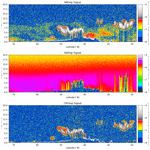# The generation of EarthCARE L1 test data sets using atmospheric model data sets

David P. Donovan, Pavlos Kollias, Almudena Velázquez Blázquez, and Gerd-Jan van Zadelhoff
Abstract

The Earth Cloud, Aerosol and Radiation Explorer mission (EarthCARE) is a multi-instrument cloud–aerosol–radiation process study mission embarking a high spectral resolution lidar, a cloud profiling radar, a multi-spectral imager, and a three-view broadband radiometer. An important aspect of the EarthCARE mission is its focus on instrument synergy. Many L2 products are the result of L1 inputs from one or more instruments. Since no existing complete observational proxy data sets comprised of co-located and co-temporal “EarthCARE-like” data exists, it has been necessary to create synthetic data sets for the testing and development of various retrieval algorithms and the data processing chain. Given the synergistic nature of the processing chain, it is important that the test data are physically consistent across the various instruments. Within the EarthCARE project, a version of the EarthCARE simulator multi-instrument framework (ECSIM) has been used to create unified realistic test data frames. These simulations have been driven using high-resolution atmospheric model data (described in a companion paper). In this paper, the methods used to create the test data scenes are described. In addition, the simulated L1 data corresponding to each scene are presented and discussed.

Share
Dates
1 Introduction

The Earth Cloud, Aerosol and Radiation Explorer (EarthCARE; ) platform comprises a 94 GHz Doppler cloud profiling radar (CPR), 355 nm high spectral resolution atmospheric lidar (ATLID), a multispectral imager (MSI), and a three-view broadband radiometer (BBR). The mission is complex in several aspects. In particular, the synergistic nature of many of the data products and the end-to-end nature of the processing chain gives rise to the requirement to generate test data which are realistic and physically consistent from the point of view of all the instruments. For example, combined ATLID, CPR, and MSI measurements will be used within the same synergistic algorithm in order to retrieve, e.g., cloud particle size information. Thus, it is important, from the point of view of the various instruments, that the simulations be conducted in a physically consistent and accurate manner. Crude instrument specific parameterizations (e.g., empirical radar reflectivity vs. cloud ice water content (Ze vs. IWC) relationships) should be avoided since they are not explicitly related to the basic physical atmospheric properties in a way that can be connected to the other instruments.

The requirement to generate physically consistent simulations with all of the virtual instruments “seeing” the same atmosphere in terms of both macro- and micro-physical considerations was a prime motivation in the original development of the EarthCARE simulator (ECSIM)1 framework and its component models. ECSIM has been applied to a set of high-resolution cloud-resolving atmospheric model data in order to generate realistic L1 test data sets suitable for EarthCARE algorithm development and testing. Here L1 refers to calibrated and instrument-corrected output generated by the instruments (e.g., lidar-attenuated backscatter, radar reflectivity). L2 data refer to geophysical quantities retrieved using L1 data (e.g., optical extinction, target classification, water content).

The focus of this paper is on the general description of the L1 data sets and how they were generated rather than, e.g., specific algorithm and instrument sensitivity study results. This paper thus serves as a central reference for the several other instrument- and algorithm-specific papers belonging to the EarthCARE level 2 algorithms and data products special issue that rely on the ECSIM-generated L1 test data sets. This paper first presents an overview of the ECSIM. Subsequently, the specific radiative-transfer and instrument simulation methods used for each of the instruments is presented. The paper then concludes with a presentation of the simulated L1 data of the three main EarthCARE testing scenes. The input data used to build these testing scenes are described in a companion paper .

2 ECSIM overview

ECSIM is not a single model, but rather it is a collection of tools, including radiative-transfer models and instrument models, that cooperate to produce physically consistent simulations covering a range of diverse instruments.

Unlike many common radiative-transfer models, ECSIM is, in principle, not tied to any particular size distribution representation. In particular, multi-wavelength, particle-size-resolved optical and physical characteristics (e.g., mass, maximum dimension, extinction coefficient, and phase function) are stored in a database, while the corresponding bin-resolved size distribution information is specified in separate size distribution files. A separate master scene file stores the 3D fields of temperature, pressure, velocity, and the concentrations of relevant atmospheric gases. This structure allows for the efficient generation of the optical properties necessary to drive diverse forward models. This process is schematically depicted in Fig. 1. In the particular application described in this paper, namely the creation of test frames using Global Environmental Multi-scale (GEM) input fields as a base and supplemented by aerosol data from Copernicus Atmosphere Monitoring Service (CAMS) reanalysis fields , the GEM and CAMS data were translated into ECSIM scene files using purpose built computer programs.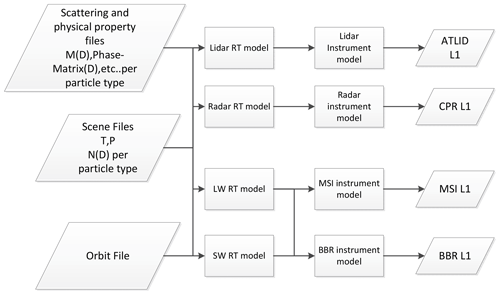Figure 1High-level structure of ECSIM. All of the radiative-transfer models read the same scene information. Another feature is that the radiative-transfer models are separate from the instrument simulation models.

A key aspects of the ECSIM structure is that all the instrument simulations are driven by the same unambiguously defined atmosphere. All of the individual radiative-transfer models read the same scene files and access the same scattering information data files. Whenever a model needs to calculate, for example, cloud extinction at a required wavelength, the appropriate binned size distribution (read from the scene files) is combined with the appropriate scattering properties (read from the scattering library). This structure facilitates the production of physically consistent simulations; i.e., it helps ensure that the radiative-transfer models all “see the same atmospheric particles” and are “discouraged” from making their own ad hoc assumptions regarding particle size distributions or optical and physical properties (e.g., different mass–maximum dimension relationships for ice cloud particles).

## 2.1 Representation of the scene constituents

### 2.1.1 Hydrometeor microphysical properties

The Global Environmental Multi-scale (GEM) Numerical Weather Prediction (NWP) models runs for the EarthCARE algorithm development efforts supports six different hydrometeor types: cloud droplets, raindrops, ice, snow, graupel, and hail using a bin microphysical representation. The model bin warm microphysics scheme represents the cloud droplets particle size distribution in 50 bin sizes centered at values corresponding to cloud droplet diameters from 2 to 100 µm and the rain particle size distribution in 50 bin sizes centered at values corresponding to raindrop radius from 50 to 2500 µm. The Environment and Climate Change Canada (ECCC) cold microphysics scheme used by GEM supports four different types of particles: cloud ice, snow, graupel, and hail. The particle size distribution of cloud ice is represented in 50 bin sizes centered at values corresponding to ice particle radius from 2 to 100 µm every 2 µm. The particle size distributions of snow is represented by 100 bin sizes each from 25 to 2500 µm every 10 µm. Table 1 summarizes the details of the bin representation of the hydrometeor species in GEM. When translating the GEM cloud information to ECSIM, certain adjustments were made to the cold cloud microphysics. As detailed in these adjustments improved the realism of the simulations. Accordingly, the ECSIM ice and snow hydrometeors categories have particle mass (M) and cross-sectional area (Ac) according to the relations for synoptic cirrus and temperatures between −40 and −20C. The mass of the ice and snow particles in grams is given by the expression $M\left(D\right)=\mathrm{exp}\left[{a}_{\mathrm{0}}+{a}_{\mathrm{1}}\mathrm{ln}\left(D\right)+{a}_{\mathrm{2}}\left[\mathrm{ln}\left(D\right){\right]}^{\mathrm{2}}\right]$, where D is in centimeters and the coefficients have values of ${a}_{\mathrm{0}}=-\mathrm{6.72924}$, a1=1.17421, and ${a}_{\mathrm{2}}=-\mathrm{0.15980}$.

### 2.1.2 VIS-UV-IR hydrometer optical properties

In ECSIM, the optical scattering properties of cloud droplets and raindrops for visible (VIS), ultra-violet (UV), and infrared (IR) wavelengths are all calculated using Mie theory. The optical scattering and absorption properties of each bin are determined by averaging the scattering and absorption properties over 20 equally spaced sub-bins. Temperature-dependent refractive indices were used and calculations were done for temperatures of 240 and 300 K and optical information at specific temperatures was found via interpolation.

For the GEM scenes, the UV-IR extinction, absorption, and phase functions for ice and snow were adapted from using the single-particle effective radius to interpolate in particle size. Here, the effective radius is defined as

$\begin{array}{}\text{(1)}& {R}_{\mathrm{eff}}\left(D\right)=\frac{\mathrm{3}M\left(D\right)}{\mathrm{4}{\mathit{\rho }}_{\mathrm{i}}{A}_{\mathrm{c}}\left(D\right)},\end{array}$

where D is the particle maximum dimension, M(D) is the particle mass, Ac(D) is the cross-sectional area of the particle, and ρi is the density of solid ice. The M(D) and Ac(D) relationships used are listed in Table 1 and are described in more detail in .

For both cloud ice and snow particle types, the Baum Aggregated Solid Columns properties were used. For cloud ice, particle maximum dimensions between 4 and 200 µm were used in steps of 4 µm. For snow, sizes between 50 and 5000 µm in steps of 25 µm were used. The aggregated solid-column phase functions generally do not have strong halo features, which is in accordance with observations . The aggregated solid-column phase functions also lack a backscatter peak; however, this is likely not in accordance with observations. In particular, in , the backscatter issue was investigated. They found that by directly solving Maxwell's equations numerically, a narrow backscattering peak was present for a wide range of types of ice crystals (i.e., irregular smoothed, smoothed, roughened hexagons). The width of the backscatter peak is inversely proportional to the size parameter. They also showed that accounting for this peak produces more realistic values of the lidar ratio and improves the agreement between lidar multiple-scattering coefficients derived using CALIPSO observations and theory . Accordingly, for the lidar specific radiative-transfer calculations (Sect. 2.2) a backscatter peak was added to the ice-phase function following the procedure described in .

For graupel and hail, for the UV-IR optical properties Mie calculations were applied to equivalent effective radius spheres. This procedure produces reasonably accurate estimates of the extinction and absorption ; however, the phase functions will not be accurate. This was not considered to be an important issue as, in the scenes considered here, occurrences of these scattering types are masked by significant amounts of cloud ice and/or snow when viewed from above.

### 2.1.3 Microwave scattering and absorption

Mie scattering is used for the estimation of the backscattering and extinction coefficients for cloud droplets and raindrops. For ice and snow, the self-similar Rayleigh–Gans approximation is used for modeling of backscattering properties of ice and snow. The particles are assumed to be horizontally aligned with an aspect ratio of 0.6. The parameters for aggregates of bullet rosettes are as in . Graupel and hail are modeled as the equal-mass spheres consisting of a blend of ice and air and using Mie theory. The dielectric properties of the mixture are calculated using Maxwell–Garnett approximation and assuming the ice inclusions in an air matrix. The gaseous attenuation is estimated using the Rosenkranz's method . The raindrops terminal velocity is estimated using and the ice and snow particle fall velocity is based on .

### 2.1.4 Aerosol microphysical and optical properties

The aerosol fields were constructed using CAMS aerosol component fields mapped to the following “Hybrid End-To-End Aerosol Classification” (HETEAC) base component types following Table 2; more detail is given in . We reiterate here that goal of this exercise is not to produce an “optimally accurate” mapping. An ad hoc approximate approach in order to produce a range of “realistic enough” aerosol conditions with different optical characteristics was sufficient for the goals of the work presented here.

Table 2Mapping between CAMS aerosol components and HETEAC aerosol component types. The CAMS fields used are desert dust (DD) size ranges 1 to 3, sea salt (SS) sizes ranges 1 and 2, sulfate (SO4), hydrophilic black carbon (BCB), and hydrophilic organic matter (OMB).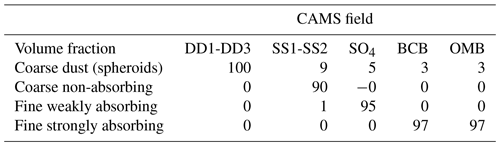### 2.1.5 Optical gaseous absorption

The ECSIM scenes contain the following (limited) number of gaseous species: O2, N2, CO2, H2O, O3.

For the MSI and BBR simulations, the atmospheric transmission is calculated using a correlated-k method for various narrow bands. For each narrow band, the aerosol and cloud optical properties are treated as being constant and represent a simple average across the band.

### 2.1.6 Shortwave surface radiative properties

For the shortwave water surfaces, the azimuth-dependent bi-directional reflectance distribution function (BRDF) for the GEM derived scenes were calculated as a function of wind speed based on lookup tables derived from the matrix operator model (MOMO) radiative-transfer model (run in unpolarized mode). The MOMO ocean BRDFs themselves are based on a Cox–Munk approach.

For the non-snow and ice SW land surfaces, the isometric–volumetric–geometric (Iso-Vol-Geo) kernel-based approach is used . For the GEM scenes, Iso-Vol-Geo coefficients for non-ice and snow areas for a number of specific bands were supplied. The BRDFs at these bands then served as inputs to a principal-component-based procedure for generating BRDFs for general SW frequencies . For ice and snow surfaces, the surfaces were treated as being Lambertian with the albedo being assigned by underlying surface type (taken from the GEM model) following .

### 2.1.7 Longwave surface radiative properties

For the longwave radiative-transfer calculations a much simpler approach was used. The surfaces were treated as being Lambertian, and the emissivity was assumed to be a constant value of 0.93 over water and 0.98 over land.

The lidar simulation is separated into two main steps. First a lidar-specific radiative-transfer model is used to calculate the time-spectral-polarization-resolved lidar backscatter. Then a separate instrument model is applied to the output of the lidar radiative-transfer model to simulate the effect of, e.g., instrument noise and non-ideal spectral filtering. The lidar radiative-transfer methods are described in this section, and the lidar instrument model is then described in Sect. 2.3.

### 2.2.1 Basic considerations

For a single-wavelength non-polarized lidar, the single-scattered power incident upon the detection element for a completely elastic backscatter lidar (neglecting the background signal) for an appropriate wavelength interval centered around λl can be written as

$\begin{array}{}\text{(2)}& P\left(z,{\mathit{\lambda }}_{\mathrm{l}}\right)={C}_{\mathrm{lid}}{z}^{-\mathrm{2}}{\mathit{\beta }}_{\mathit{\pi }}\left(z,{\mathit{\lambda }}_{\mathrm{l}}\right)\mathrm{exp}\left[-\mathrm{2}\underset{\mathrm{0}}{\overset{z}{\int }}\mathit{\alpha }\left({z}^{\prime },{\mathit{\lambda }}_{\mathrm{l}}\right)\mathrm{d}{z}^{\prime }\right]\phantom{\rule{0.125em}{0ex}},\end{array}$

where λl is the laser wavelength, P(z,λl) is the power the lidar will receive from a target a distance $z=ct/\mathrm{2}$ from the lidar (where t is the elapsed time since the laser pulse was launched from the lidar), βπ(z,λl) is the range- and wavelength-dependent backscatter coefficient, and $\mathit{\alpha }\left({z}^{\prime },{\mathit{\lambda }}_{\mathrm{l}}\right)$ is the extinction coefficient. Here both the backscatter and extinction coefficients represent appropriate averages over the wavelength interval of interest. Clid is a constant that takes into account factors related to the lidar's physical characteristics.

$\begin{array}{}\text{(3)}& {C}_{\mathrm{lid}}={T}_{\mathrm{r}}{E}_{\mathrm{l}}{A}_{o},\end{array}$

where Tr is the bulk effective receiver transmission, El is the laser pulse energy, and Ao is the effective receiver area.

### 2.2.2 Multiple scattering

Equation (2) is valid only when single scattering is predominant. In cases where either a high proportion of scattered photons remain within the receiver field of view (FOV) or the optical depth is such that photon mean free path is small compared to the instantaneous sampling volume then multiple scattering (MS) effects must be taken into account. In general, these conditions are often met for ground-based remote sensing of clouds and are always met for the case of space-based cloud remote sensing.

In order to account for multiple scattering, the lidar radiative-transfer simulations were conducted using the ECSIM-3D lidar Monte Carlo radiative transfer code. The approach used is described within , and additional relevant detail not covered within is given here. In particular, the approach used to determine the spectral properties of the return signal is discussed. Within the MS model, each photon packet is assigned both a mean relative spectral shift (initially zero) from its center wavelengths and a Gaussian spectral width. The Doppler shift in the packets is calculated according to the relative velocity of the scatterers encountered and the scattering geometry. The spectral width is initialized using the laser line width and is hardly affected by particulate scattering but can be substantially widened if molecular scattering has occurred.

### 2.2.3 Doppler shift and multiple scattering

Even neglecting such processes involving the change in energy levels within the target scatterers (i.e., rotational–vibrational Raman scattering, fluorescence) the scattered radiation will be Doppler-shifted according to the relative motion of the scatterers with respect to the lidar. In general, a target moving with respect to a fixed lidar will “see” a source whose frequency is given by (neglecting terms of order ${\left(v/c\right)}^{\mathrm{2}}$)

$\begin{array}{}\text{(4)}& f={f}_{o}\left(\mathrm{1}+\frac{v}{c}\mathrm{cos}\left(\mathit{\theta }\right)\right)\phantom{\rule{0.125em}{0ex}},\end{array}$

where v is the velocity of the scatterer, c is the speed of light, θ is the angle between the scatterers velocity vector and the line-of-sight directed back toward the receiver, and fo is the frequency of the source light in the frame of the laser. When light initially incident upon the moving scatterer, is scattered, it is equivalent to being instantaneously absorbed and re-emitted so that the scattered light will again be Doppler shifted in frequency depending on the motion of the scatterer with respect to the receiver. Accordingly, the frequency measured by an observer in the same frame as the lidar transmitter will be given by (again neglecting terms of order ${\left(v/c\right)}^{\mathrm{2}}$)

$\begin{array}{}\text{(5)}& f={f}_{o}\left[\mathrm{1}+\frac{v}{c}\left(\mathrm{cos}\left(\mathit{\theta }\right)+\mathrm{cos}\left({\mathit{\theta }}_{\mathrm{sc}}\right)\right)\right]\phantom{\rule{0.125em}{0ex}},\end{array}$

where θsc is the angle between the velocity vector and the scattered photon's trajectory. This means that light scattered directly forward and measured by a stationary observer would have no Doppler shift while light scattered directly backwards to the lidar would be observed to have been shifted in wavelength by an amount twice that predicted by Eq. (4).

The occurrence of multiple scattering will have implications with respect to the spectral signature of the lidar return. By repeated application of Eq. (4) (see Fig. 2) it can be shown that the Doppler-shifted frequency after n scatters as measured by an observer in the same frame of reference as the lidar will be given by

$\begin{array}{}\text{(6)}& \begin{array}{rl}{f}_{n}=& {f}_{o}\left(\mathrm{1}+\frac{\mathrm{1}}{c}\left({\mathbit{v}}_{\mathrm{1}}\cdot {\mathbit{I}}_{\mathrm{0}}+\left({\mathbit{v}}_{\mathrm{1}}-{\mathbit{v}}_{\mathrm{2}}\right)\cdot {\mathbit{I}}_{\mathrm{1}}\\ & +\left({\mathbit{v}}_{\mathrm{2}}-{\mathbit{v}}_{\mathrm{3}}\right)\cdot {\mathbit{I}}_{\mathrm{2}}+\mathrm{\cdots }{\mathbit{v}}_{n}\cdot {\mathbit{I}}_{n}\right)\right)\phantom{\rule{0.125em}{0ex}},\end{array}\end{array}$

where vi is the velocity vector of the ith scatterer and Ii is the unit vector for the photon trajectory for the ith scatter. If the lidar is to observe the photon to a good approximation, it must be scattered directly backwards towards the lidar receiver so that ${\mathbit{I}}_{n}=-{\mathbit{I}}_{\mathrm{0}}$ and thus

$\begin{array}{}\text{(7)}& \begin{array}{rl}{f}_{n}=& {f}_{o}\left(\mathrm{1}+\frac{\mathrm{1}}{c}\left({\mathbit{v}}_{\mathrm{1}}\cdot {\mathbit{I}}_{\mathrm{0}}+\left({\mathbit{v}}_{\mathrm{1}}-{\mathbit{v}}_{\mathrm{2}}\right)\cdot {\mathbit{I}}_{\mathrm{1}}+\\ & \left({\mathbit{v}}_{\mathrm{2}}-{\mathbit{v}}_{\mathrm{3}}\right)\cdot {\mathbit{I}}_{\mathrm{2}}+\mathrm{\cdots }-{\mathbit{v}}_{n}\cdot {\mathbit{I}}_{\mathrm{0}}\right)\right)\end{array}.\end{array}$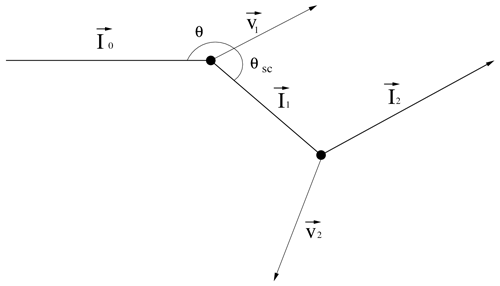Figure 2Geometry relevant for determining the observed Doppler shift after multiple scatters.

### 2.2.4 Spectral broadening and multiple scattering

In the MC model the spectral width of the packets is also tracked. The width is initialized using the laser spectral line width. The Gaussian width after subsequent scattering events is calculated by convolving the spectral width of the incoming packet with the spectral width induced by the scattering event (accounting the scattering geometry). For particulate scattering, the spectral width is assumed to be unaffected. However, for molecular scattering events thermal broadening is important. How this is modeled in ECSIM is described in the following subsection.

A schematic representation of the spectral signature of a general lidar return signal is shown in Fig. 3. The spectral width of the particulate backscattering peak will be determined by the spectral width of the laser pulse itself along with any turbulence present in the sampling volume. The spectral width of the laser will be on the order of 10−7fo, so that the laser line width will usually be the dominant factor. The molecular backscatter though, will be much spectrally broader than the particulate scattering return; this is due to the fact that the atmospheric molecules have a large thermal velocity. For low densities the half-maximum half width (HMHW) of the broadening produced by the thermal motions alone is given by

$\begin{array}{}\text{(8)}& \mathrm{\Delta }f={f}_{o}\left(\frac{\mathrm{8}kT\mathrm{log}\left(\mathrm{2}\right)}{M{c}^{\mathrm{2}}}\right),\end{array}$

where k is Boltzmann's constant, M is the average molecular mass, and T is temperature. For typical values of atmospheric temperature, the HMHW of the Doppler return will be on the order of $\mathrm{4}×{\mathrm{10}}^{-\mathrm{6}}{f}_{o}$ so that the Doppler broadening will be 10–20 greater than the laser line width. Equation (8) applies at low gas densities.

In general, the central (non-Raman) Rayleigh line profile (Cabannes line) will consist of three components, a central peak together with two flanking “Brillouin–Mandel'shtam” peaks . In the low-density or high-temperature regimes the uncorrelated motion of the scatterers gives rise to a Gaussian velocity distribution centered around the mean velocity of the flow and Eq. (8) applies. As the pressure increases or the temperature decreases, density fluctuations on the order of the laser wavelength will appear. These density fluctuations travel at the speed of sound in the gas and will give rise to acoustic side bands.

The Rayleigh–Brillouin scattering line shape may be quantified in terms of the so-called y parameter, which is defined in terms of the ratio of the laser wavelength to the mean free path. For the Earth's atmosphere

$\begin{array}{}\text{(9)}& y=\mathrm{0.230}\left[\frac{T+\mathrm{111}}{{T}^{\mathrm{2}}}\right]\left[\frac{P\mathit{\lambda }}{\mathrm{sin}\left(\frac{\mathit{\theta }}{\mathrm{2}}\right)}\right]\phantom{\rule{0.125em}{0ex}},\end{array}$

where T is the temperature in Kelvin, P is the pressure in atmospheres, λ is the laser wavelength in nanometers, and θ is the scattering angle. Here x is a normalized frequency parameter defined as

$\begin{array}{}\text{(10)}& x=\frac{\sqrt{\mathrm{2}}\mathit{\lambda }}{\mathrm{4}\mathrm{sin}\left(\frac{\mathit{\theta }}{\mathrm{2}}\right)}\left(\frac{\mathit{\nu }}{{\mathit{\nu }}_{o}}\right),\end{array}$

where ν is the frequency shift from the line center and νo is the sound speed

$\begin{array}{}\text{(11)}& {\mathit{\nu }}_{o}=\sqrt{\frac{kT}{m}},\end{array}$

where m is the molecular mass.

For low y parameters, the line shape has a simple Gaussian form. For larger values of y (in the range that we must be concerned with) the line shape becomes complicated and somewhat costly to evaluate. Fortunately, for values of y under about 10, the Rayleigh–Brillouin line shape may be accurately approximated by the sum of three independent Gaussian functions (Witschas2011).

For the single-scatter return the line shape is determined by the sum of three Gaussian profiles. These calculations are based on the results of pre-computed approximate Gaussian fits made to accurate calculations of the line profile corresponding to various y parameters (see Fig. 4). For the higher-order scattering, at each molecular scattering event the probability of the scattered photon packet being shifted to one of the acoustic side bands is calculated. Whether or not the center frequency of the photon packet is shifted is then determined stochastically using the relative weights of the component Gaussians, and the subsequent shift and width of the associated Gaussian profile is used to determine the line shape. This line shape and shift is then used subsequently for the next multiple-scattering event.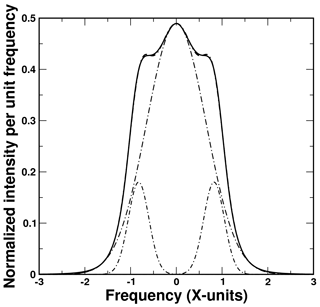Figure 4Exact Rayleigh–Brillouin line shape (solid line) along with fitted sum of three Gaussian functions (dashed–dotted line). The three-component Gaussian functions are also shown. Here the y parameter is equal to 1.0.

## 2.3 Lidar instrument model

ATLID transmits a linearly polarized beam and possesses three receiver channels. A co-polar “Mie” channel which detects mainly particulate (i.e.,  clouds and aerosol) backscatter, a co-polar “Rayleigh” channel which detects mainly backscatter from molecules, and a cross-polar particulate+molecular channel. The Mie-Rayleigh separation is achieved using a Fabry–Pérot etalon. For details of ATLID's design, see and . Some of the important ATLID technical specifications are repeated in Table 3.

The ECSIM lidar instrument model ingests the output of the lidar radiative transfer model and models the instrument response function including instrument noise. The model is capable of being configured to simulate various lidars, in this case ATLID. The instrument model ingests the time-spectral-polarization-resolved output of the lidar radiative transfer model and models the three different optical ATLID detection channels. Explicit modeling of the Fabry–Pérot etalon high-spectral resolution lidar (HSRL) spectrometer and various other optical elements in each detector path are performed. The effects of Poisson shot noise on the simulated observed signals is simulated. Instrument dark current and solar background effects are taken into account. Since ATLID's detector elements are accumulation charge-coupled devices (ACCDs) they are subject to read-out noise. The effects of this read-out noise are also simulated.

### 2.3.1 Background signal

The background signal refers to power registered by the lidar receiver that is due to the detection of photons from sources other than backscattered laser light. In the case here, the main source of background light will be scattered sunlight from the Earth's surface and atmosphere. As such, the background will depend on the solar angle, the surface type, and the cloud cover. In this work, the lidar background values were based on an approximate lookup table approach where the background irradiance at 355 nm was modeled using a radiative model for various cloud optical depths, surface albedos, and solar zenith angles.

The background power incident upon the detector level is given by

$\begin{array}{}\text{(12)}& {P}_{\mathrm{back}}={A}_{o}\mathit{\pi }{\mathit{\rho }}_{\mathrm{t}}\stackrel{\mathrm{‾}}{{I}_{b}\left(\mathit{\lambda }\right){T}_{\mathrm{rec}}\left(\mathit{\lambda }\right)}\mathrm{\Delta }\mathit{\lambda },\end{array}$

where Ao is the effective receiver area, ρt is the telescope 0.5 angle field of view, $\stackrel{\mathrm{‾}}{{I}_{b}\left(\mathit{\lambda }\right){T}_{\mathrm{rec}}\left(\mathit{\lambda }\right)}$ is the average product of the receiver-wavelength-dependent transmission (including filtering) with the upwelling irradiance, and Δλ is the wavelength interval that must be considered.

The ATLID lidar receiver optical train is modeled as a sequence of elements (beam splitters, spectral filters, half-wave plates, etc.) that operate on the spectral and polarization state of the lidar return. For simplicity, broadband spectral filters are modeled as having a rectangular passbands and are characterized by a single in-band transmission and reflection and an out-of-band reflection and transmission pair.

The Fabry–Pérot (FP) etalon is the most critical optical element in ATLID's receiver chain. This element is used to separate the Mie signal from the Rayleigh signal.

The transmission function of an etalon without accounting for non-ideal effects such as the finite input beam collimation and surface roughness may be modeled as

$\begin{array}{}\text{(13)}& T=\left(\mathrm{1}-\frac{A}{\mathrm{1}-R}\right)×\frac{\mathrm{1}}{\mathrm{1}+\frac{\mathrm{4}R}{\left(\mathrm{1}-R{\right)}^{\mathrm{2}}}{\mathrm{sin}}^{\mathrm{2}}\frac{\mathit{\delta }}{\mathrm{2}}}\phantom{\rule{0.125em}{0ex}},\end{array}$

where R is the reflection coefficient of the etalon mirrors, A is the relative absorption and scattering loss parameter, and $\mathit{\delta }=\mathrm{2}\mathit{\pi }\frac{\mathit{\lambda }-{\mathit{\lambda }}_{o}}{{\mathit{\lambda }}_{o}}$, where λo is the central wavelength. The corresponding etalon reflection function (Rfp) may be written as follows:

$\begin{array}{}\text{(14)}& {R}_{\mathrm{fp}}=\frac{{\left(\mathrm{1.0}-R-A\right)}^{\mathrm{2}}}{\mathrm{1.0}-\mathrm{2.0}×R\mathrm{cos}\left(\mathit{\delta }\right)+{R}^{\mathrm{2}}}\phantom{\rule{0.125em}{0ex}}.\end{array}$

The effects of non-parallel mirror flatness, diffraction, and non-ideal beam collimation are taken into account by convolving the above absorbing etalon transmission and reflection functions by a top-hat function whose width in wavenumbers is given by

$\begin{array}{}\text{(15)}& \mathrm{\Delta }{\mathit{\nu }}_{\mathrm{total}}=\sqrt{\mathrm{\Delta }{\mathit{\nu }}_{\mathrm{np}}^{\mathrm{2}}+\mathrm{\Delta }{\mathit{\nu }}_{\mathrm{ap}}^{\mathrm{2}}+\mathrm{\Delta }{\mathit{\nu }}_{\mathrm{dif}}^{\mathrm{2}}},\end{array}$

where Δνnp accounts for the broadening due to non-parallel mirror alignment, Δνap accounts for collimation or finite aperture effects

$\begin{array}{}\text{(16)}& \mathrm{\Delta }{\mathit{\nu }}_{\mathrm{ap}}=\mathrm{1.0}-\mathrm{cos}\left[{\left(\frac{{D}_{\mathrm{t}}}{{D}_{\mathrm{fp}}}\right)}^{\mathrm{2}}\frac{{\mathit{\rho }}_{\mathrm{t}}}{\mathrm{2}}\right]\phantom{\rule{0.125em}{0ex}},\end{array}$

where Dt is the telescope diameter, Dfp is the etalon diameter, and ρt is the telescope full-angle field of view in radians. Δνdif accounts for diffraction effects and is given by

$\begin{array}{}\text{(17)}& \mathrm{\Delta }{\mathit{\nu }}_{\mathrm{dif}}=\mathrm{1.0}-\mathrm{cos}\left[\left(\frac{{\mathit{\lambda }}_{o}}{{D}_{\mathrm{t}}}\right)\right].\end{array}$

An example etalon transmission and reflection profile as a function of ννo is shown in Fig. 5. Here T=0.978, A=0.0, the free spectral range (the distance between adjacent transmission maximums) is 0.5 cm−1, the flatness parameter is ${\mathit{\lambda }}_{o}/\mathrm{300}$, the telescope field of view (FOV) is $\mathrm{5}×{\mathrm{10}}^{-\mathrm{3}}$ mrad, the telescope diameter is 0.6 m, and the etalon diameter is 0.05 m.

### 2.3.3 Noise considerations

For a given time interval, the number of photons arriving at a given detector channel is given by

$\begin{array}{}\text{(18)}& {N}_{\mathrm{det}}={R}_{\mathrm{det}}\frac{{\mathit{\lambda }}_{o}}{hc}\left({P}_{\mathrm{lid}}+{P}_{\mathrm{back}}\right)\mathrm{\Delta }t\phantom{\rule{0.125em}{0ex}},\end{array}$

where Δt is the averaging time interval, h is Planck's constant, λo is an appropriate mean wavelength for the detector channel in question, Rdet is the detector quantum efficiency, Plid is the power received at the lidar detector, and Pback is the background power arising from the upwelling reflected solar radiance at 355 nm. According to Poisson statistics, the standard deviation of Ndet, shot noise, is simply given by

$\begin{array}{}\text{(19)}& \mathit{\delta }{N}_{\mathrm{det}}=\sqrt{{N}_{\mathrm{det}}}.\end{array}$

In addition to the shot noise, the effects of dark current noise and ACCD readout noise are also simulated. The average dark current is a characteristic of the detection electronic chain, and its mean level is assumed to be constant in terms of effective noise photocounts per unit time. The dark-count rate is assumed to follow Poisson statistics. The read-out noise is a characteristic of CCD detectors and is applied only at simulated read-out time. For ATLID, the envisioned default mode is for the ACCD to accumulate two shots before read-out. The mean read-out noise is assumed to be constant per read-out event and is modeled using Poisson statistics.

### 2.3.4 Spectral and polarization cross-talk

The characteristics of the HSRL filter ensure that a degree of spectral cross-talk between the Mie and Rayleigh channels exists . The effects of cross-talk are simulated within the lidar instrument model. The lidar instrument model also applies a cross-talk correction, following the ATLID L0 to L1 processor step. This correction assumes perfect knowledge of the appropriate cross-talk coefficients. In flight, uncertainties in the coefficients will introduce additional errors; however, this is not envisioned to be a significant issue as the in-flight values of the coefficients are envisioned to be known within an accuracy of a few percent. This is to be achieved using a combination of a priori on-ground characterization and in-flight monitoring and adjustment, e.g., using returns above 30 km (where only molecular scattering returns should be expected) to quantify the amount of Rayleigh-to-Mie cross-talk and using ground and suitably strong cloud returns (where to a good approximation only elastic particulate scattering returns exist) to quantify the Mie-to-Rayleigh cross-talk. Due to the occurrence of variable but significant amounts of molecular Brillouin scattering in liquid water , water surfaces are not expected to be suitable cross-talk coefficient determination targets for ATLID.

For simplicity, the simulations presented in this paper did not consider polarization cross-talk. However, as is the case with lidars in general, due to non-ideal optical element characteristics, laser polarization purity, and imperfect optical element orientation and alignments, a degree of polarization cross-talk will be present in the ATLID instrument . The correction of spectral cross-talk effects will be conducted at the L1 stage by the L0 to L1 processor. The estimated errors will be reported in the L1 product.

The characterization of the ATLID polarization cross-talk has been the subject of extensive ground testing and characterization, e.g., . For example, in-flight characterization and monitoring of the spectral cross-talk is planned to be accomplished using high-altitude returns where the Rayleigh depolarization signal is known and from solar background measurements over thick ice clouds at high sun conditions.

The radar scattering calculations described in Sect. 2.1.3 are used to estimate the radar backscatter cross-section σb,j(D) for each hydrometeor species j and particle maximum diameter D in units of m2. In addition, the extinction cross-section σe,j(D) for each hydrometeor species j and particle maximum diameter D is estimated in units of m2. The GEM particle size distribution number concentration nj(D) is provided in units of m−3 (integrated across the bin diameter width) and the particle sedimentation velocity Vj(D) is provided in units of m s−1.

Thus, the radar reflectivity factor Ze (mm6 m−3), specific attenuation A (dB km−1) and reflectivity-weighted hydrometeor sedimentation velocity VSED (m s−1) are estimated for each hydrometeor species as follows:

$\begin{array}{}\text{(20)}& {Z}_{\mathrm{e},j}={\mathrm{10}}^{\mathrm{18}}\frac{{\mathit{\lambda }}^{\mathrm{4}}}{{\mathit{\pi }}^{\mathrm{5}}|{K}_{\mathrm{w}}^{\mathrm{2}}|}\sum _{i=\mathrm{1}}^{\mathrm{Nbins}}\left[{\mathit{\sigma }}_{\mathrm{b},j}\left(D\right){n}_{j}\left(D\right)\right],\end{array}$

$\begin{array}{}\text{(21)}& {A}_{j}=\frac{\mathrm{0.01}}{\mathrm{ln}\left(\mathrm{10}\right)}\sum _{i=\mathrm{1}}^{\mathrm{Nbins}}\left[{\mathit{\sigma }}_{\mathrm{e},j}\left(D\right){n}_{j}\left(D\right)\right],\end{array}$

$\begin{array}{}\text{(22)}& {V}_{\mathrm{SED},j}=\frac{{\sum }_{i=\mathrm{1}}^{\mathrm{Nbins}}\left[{\mathit{\sigma }}_{\mathrm{b},j}\left(D\right)\phantom{\rule{0.25em}{0ex}}{n}_{j}\left(D\right)\phantom{\rule{0.25em}{0ex}}{V}_{j}\left(D\right)\right]}{{\sum }_{i=\mathrm{1}}^{\mathrm{Nbins}}\left[{\mathit{\sigma }}_{\mathrm{b},j}\left(D\right){n}_{j}\left(D\right)\right]}\phantom{\rule{0.125em}{0ex}},\end{array}$

where λ is the wavelength in m, j is the index for the hydrometeor species (cloud, rain, ice, snow, graupel, and hail), and $\mathrm{|}{K}_{\mathrm{w}}^{\mathrm{2}}\mathrm{|}$ is the dielectric factor of water at 94 GHz. The aforementioned parameters are combined to produce their total value at each GEM grid point

$\begin{array}{}\text{(23)}& {Z}_{\mathrm{e}}=\sum _{j=\mathrm{1}}^{\mathrm{6}}{Z}_{\mathrm{e},j}\phantom{\rule{0.25em}{0ex}}\phantom{\rule{0.25em}{0ex}}{A}_{\mathrm{h}}=\sum _{j=\mathrm{1}}^{\mathrm{6}}{A}_{j}\phantom{\rule{0.25em}{0ex}}\phantom{\rule{0.25em}{0ex}}{V}_{\mathrm{SED}}=\frac{{\sum }_{j=\mathrm{1}}^{\mathrm{6}}\left[{Z}_{\mathrm{e},j}{V}_{\mathrm{SED},j}\right]}{{Z}_{\mathrm{e}}}.\end{array}$

In addition to hydrometeor attenuation (Ah), gaseous attenuation (Ag) can have a significant impact on the measured CPR reflectivity. For example, two-way gaseous attenuation from the surface to the upper troposphere of more than 5 dB is not unusual in the tropics. compared different models to estimate absorption at W-band by gases by taking advantage of the colocated CloudSat–Cloud Aerosol Lidar and Infrared Pathfinder Satellite Observation (CALIPSO) measurements. Their results indicate that the model fits the observations best. The gaseous attenuation at each model grid point Ag (dB km−1) is calculated using and the water vapor mixing ratio profile from the EarthCARE auxiliary meteorological product (X-MET, ). Consistent with the nadir viewing of the EarthCARE CPR, the Ah and Ag estimates are computed assuming propagation along the GEM model vertical dimension.

The normalized (per unit of area) cross-section of the Earth's surface σ0 [m−1] is estimated. Over an ocean surface, the normalized cross-section is estimated using the relationship from as a function of the near-surface wind speed provided in the X-MET data product. CloudSat observations have shown that over the ocean surface σ0 is known within 2 dB and over land exhibits very large variability due to its dependency on vegetation, surface slope, soil moisture, snow cover, and other factors . At 94 GHz, the ocean surface σ0 varies between 16 and 6 dB for near-surface wind speeds of 2 to 20 m s−1 . The corresponding radar reflectivity factor for the Earth's surface is estimated using the following formula:

$\begin{array}{}\text{(24)}& {Z}_{\mathrm{SFC}}={\mathrm{10}}^{\mathrm{18}}\frac{{\mathit{\sigma }}_{\mathrm{0}}{\mathit{\lambda }}^{\mathrm{4}}}{{\mathit{\pi }}^{\mathrm{5}}|{K}_{\mathrm{w}}{|}^{\mathrm{2}}\mathrm{\Delta }Z}\phantom{\rule{0.125em}{0ex}},\end{array}$

where ΔZ is the EarthCARE CPR range resolution (500 m). Ze and ZSFC are intrinsic reflectivities (unattenuated). The measured (attenuated) CPR reflectivity Ze,m at a height z in the GEM model can be defined in terms of intrinsic reflectivity (unattenuated), and the hydrometeors and gaseous-specific attenuation are defined using the following formula:

$\begin{array}{}\text{(25)}& {Z}_{\mathrm{e},m}\left(z\right)={Z}_{\mathrm{e}}\left(z\right){\mathrm{exp}}^{-\mathrm{0.46}{\sum }_{{z}_{\mathrm{top}}}^{z}\left({A}_{\mathrm{h}}\left(z\right)+{A}_{\mathrm{g}}\left(z\right)\right)\mathrm{\Delta }z}\phantom{\rule{0.125em}{0ex}},\end{array}$

where ztop is the highest level in the GEM model (representing the top of the atmosphere). Note that the value of −0.46 is due to the fact that the attenuation coefficients are in dB km−1. If the height in the model is set to zero, then this formula can be used to estimate the attenuated Earth's surface radar reflectivity.

### 2.4.2 Multiple scattering

Multiple scattering (MS) signatures have been observed in spaceborne radar observations . The MS contribution to the observed spaceborne radar observations is more pronounced at higher radar frequencies and for the larger instantaneous field of view. Although the EarthCARE CPR has a smaller radar footprint compared to CloudSat, we do expect to see significant MS effects, especially in deep convective cores . Here, the fast radar multiple scattering model for wide-angle scattering using the time-dependent two-stream approximation introduced by is used to estimate the CPR multiple scattering profiles. The MS model can only provide forward-simulated MS CPR radar reflectivity. Panels (c) in Figs. 13, 21, and 28 show examples of CPR forward simulations using the MS model. We did not perform MS simulations of the CPR Doppler velocity since we plan to flag and remove the CPR profiles with significant MS contributions .

The EarthCARE CPR instrument model has two main modules. The first is the sampling geometry module that determines which part of the GEM model is sampled by the CPR at any given time step (or along-track location) and also accounts for the along-track displacement of the satellite sampling volume during signal integration. The second module is the CPR receiver module that introduces the instrument noise and estimates the CPR Doppler moments and their corresponding uncertainty . Some of the important EarthCARE CPR technical specifications are shown in Table 4.

Table 4CPR technical specifications. The last two rows refer to the beam 3 dB full width measured in degrees and the footprint on the ground.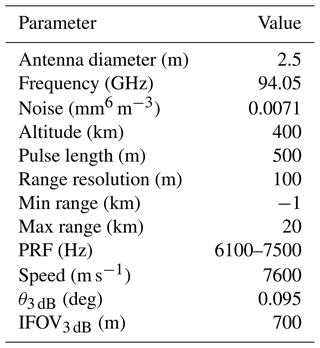Following , the antenna weighting function Wa(x,y) is shown in Fig. 6, where x and y are the along- and cross-track dimensions, respectively. The Wa(x,y) determines the contribution of GEM model grid point radar reflectivity Ze(x,y) to the total radar reflectivity observed by the CPR at a particular model grid. At a GEM model height z, the radar reflectivity contribution ZGEM(z) to the CPR is estimated using the following equation:

$\begin{array}{}\text{(26)}& {Z}_{\mathrm{GEM}}\left(z\right)=\sum _{i=\mathrm{1}}^{\mathrm{Xbins}}\sum _{j=\mathrm{1}}^{\mathrm{Ybins}}\left[{Z}_{\mathrm{e},m}\left(i,j\right){W}_{\mathrm{a}}\left(i,j\right)\right]\phantom{\rule{0.125em}{0ex}},\end{array}$

where Xbins and Ybins are the number of GEM grid points that are illuminated by the CPR radiation. Next, the CPR range weighting function Wr(x,y) that described the along range point target response of a radar is applied to estimate CPR measured radar reflectivity at a particular range r. The CPR range weighting functions for the EarthCARE and CloudSat CPRs are shown in Fig. 6.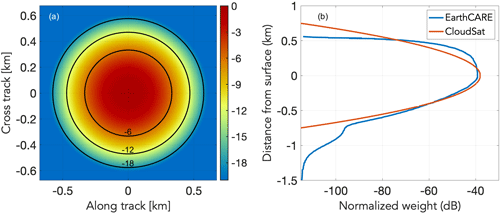Figure 6The EarthCARE CPR antenna weighting function Wa(x,y) distributions in the along- and cross-track direction (a). Values are in dB below the peak value. The range weighting function Wr(x,y) for CloudSat and EarthCARE (b) is also shown.

The CPR range weighting function is the result of the transmitted waveform (the same for both radar) and the CPR receiver filter. The EarthCARE CPR receiver filter was designed to sharply cut off the Wr(x,y) at 500 m above the Earth's surface to improve the detection of low-level clouds compared to CloudSat . The CPR radar reflectivity at range r is estimated using the following equation:

$\begin{array}{}\text{(27)}& {Z}_{\mathrm{CPR}}\left(r\right)=\sum _{i=\mathrm{1}}^{\mathrm{Gbins}}\left[{Z}_{\mathrm{GEM}}\left(z\left(i\right)-r\right){W}_{\mathrm{r}}\left(z\left(i\right)-r\right)\right]\phantom{\rule{0.125em}{0ex}},\end{array}$

where Gbins is the number of GEM model levels within the limits of the CPR range weighting function centered at range r. The forward simulation of the EarthCARE CPR Doppler velocity and spectrum width requires us to introduce the apparent Doppler velocity that the Vapp(x) introduced to each GEM model point within the CPR footprint will have due to the satellite motion Vsat. The Vapp(x) is estimated using the following equation:

$\begin{array}{}\text{(28)}& {V}_{\mathrm{app}}\left(x\right)=-x\frac{{V}_{\mathrm{sat}}}{{H}_{\mathrm{sat}}},\end{array}$

where x is the distance from nadir and Hsat is the altitude of the satellite Fig. 7. The Vapp(x) is independent of the cross-track distance y; thus, ${V}_{\mathrm{app}}\left(x,y\right)={V}_{\mathrm{app}}\left(x\right)$. At a GEM model height z, the Doppler velocity contribution VGEM(z) to the CPR is estimated using the following equation:

$\begin{array}{}\text{(29)}& \begin{array}{rl}{V}_{\mathrm{GEM}}\left(z\right)=& \sum _{i=\mathrm{1}}^{\mathrm{Xbins}}\sum _{j=\mathrm{1}}^{\mathrm{Ybins}}\left[{Z}_{\mathrm{e},m}\left(i,j\right)\phantom{\rule{0.25em}{0ex}}{W}_{\mathrm{a}}\left(i,j\right)\phantom{\rule{0.25em}{0ex}}\left({V}_{\mathrm{app}}\left(i,j\right)\\ & +{W}_{\mathrm{air}}\left(i,j\right)+{V}_{\mathrm{SED}}\left(i,j\right)\right)\right],\end{array}\end{array}$

where Wair(i,j) is the vertical air motion (negative is up) and VSED(i,j) is the total sedimentation velocity at the (i,j) GEM grid point.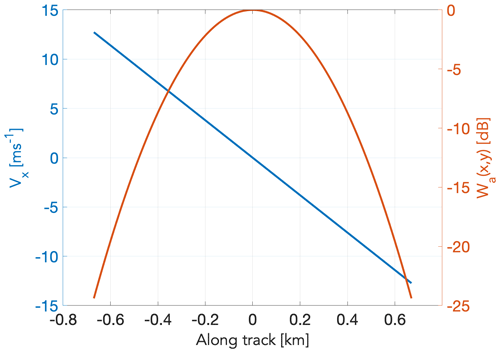Figure 7The EarthCARE CPR antenna weighting function Wa(x,y) and apparent Doppler velocity Vapp(x) distribution as a function of distance in the along-track direction

In the CPR receiver module, the product ${S}_{\mathrm{v}}\left(i,j\right)={Z}_{\mathrm{e},m}\left(i,j\right){W}_{\mathrm{a}}\left(i,j\right)$ and the sum of all the velocity components ${V}_{\mathrm{tol}}\left(i,j\right)={V}_{\mathrm{app}}\left(i,j\right)+{W}_{\mathrm{air}}\left(i,j\right)+{V}_{\mathrm{SED}}\left(i,j\right)$ are used to construct the periodogram S(V) mm6 m−3 m−1 s) of the returned radar signal at each height z of the GEM model following the methodology proposed first by . Since we are using radar reflectivity at power, the noise is also given in radar reflectivity units (see Table 3). The periodogram is a very useful tool for describing a time series data set. In a radar system, the pulse repetition frequency (PRF) determines the temporal resolution of the time series data at a particular range gate. The periodogram is an estimate of the spectral density of a signal. The periodogram is interpolated at a spectral velocity resolution that is determined by the CPR sampling rate (PRF), which determines the higher sampled frequency. This is often called Nyquist frequency (${F}_{\mathrm{N}}=\text{PRF}/\mathrm{2}$), which is half the sampling frequency of a discrete signal processing system. It is sometimes known as the folding frequency of a sampling system. Using the radar wavelength λ (Table 3), we can convert the folding frequency to folding velocity or Nyquist velocity $\left({V}_{\mathrm{N}}=\text{PRF}\mathit{\lambda }/\mathrm{4}\right)$. If the Doppler velocity of a radar target exceeds the magnitude of the Nyquist velocity, folding occurs (aliasing).

Once the radar receiver noise is added in the frequency domain, the next step is to perform an inverse fast Fourier transform (IFFT) of the constructed Doppler spectrum in order to retrieve I (in-phase) and Q (quadrature-phase) voltage time series . The in-phase channel includes the portion of the signal in phase with the reference sinusoid. The quadrature-phase channel includes the portion of the signal 90 out of phase with the reference sinusoid. The IFFT operator is applied to the amplitude spectrum. The temporal spacing of the I and Q voltages is $\mathrm{1}/\text{PRF}$. As described in , the I and Q time series at each GEM model height z are convoluted along range with the square root of the range weighting function Wr(x,y) and are then used as input to a pulse-pair Doppler moments estimator (Zrnic1977) to provide estimates of the CPR radar reflectivity, Doppler velocity, and Doppler spectrum width.

## 2.5 Multi-spectral imager (MSI) simulations

### 2.5.1 Shortwave radiative transfer calculations

The shortwave radiances for the first four EarthCARE multi-spectral imager (MSI) channels were performed by applying a one-dimensional radiative transfer model. ECSIM contains an option to perform 3D Monte Carlo calculations; however, it was unfeasible to apply this option for the totality of the domain required. The 1D calculations used DISORT with 32 streams driven by the atmospheric absorption, phase functions, and surface BRDFs extracted from the scene file. The band limits and relevant atmospheric gases for the MSI SW bands are listed in Table 5 and are based on an early instrument specification, e.g., . Here, for simplicity a top-hat response was assumed, and it should be noted that the exact band widths and centers differ from those of the actual instrument. The MSI retrieval algorithms make these same assumptions when applied to the simulated data; however, the detailed non-ideal spectral response of each channel will be fully accounted for when these algorithms are supplied with actual observations.

Table 5Shortwave multispectral imager (MSI) bands; v1 and v2 are the effective band lower and upper wavenumber limits, respectively.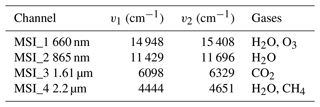Even with a 1D approach, the computation covering the 6000×150 km frame domain at a horizontal resolution of 0.25 km is computationally demanding. In order to speed up the process, a sampling and interpolation process was implemented as follows.

• 1.

The domain was divided into a number of sub-domains of n×m pixels. In this work these were n=12 and m=12.

• 2.

For each pixel in the sub-domain, the quantities ${X}_{\mathrm{1}}\left(i,j\right)={A}_{\mathrm{s}}\left(i,j\right)\mathrm{exp}\left(-\mathit{\tau }\left(i,j\right)\right)$ and ${X}_{\mathrm{2}}\left(i,j\right)=\mathrm{exp}\left(-\mathit{\tau }\left(i,j\right)\right)$ were calculated, where τ is the particulate optical depth and As is the pixel black-sky albedo.

• 3.

Within each subdomain, the pixels where DISORT would be applied to were selected using the following method:

• a.

the lower left pixels were selected by default;

• b.

in the along-track direction, each ith and jth pixel are selected by default (in this work, every fifth pixel was used);

• c.

the pixels along the spacecraft nadir track are selected by default;

• d.

the pixels with the maximum and minimum values of X1 and X2, respectively, are selected.

• 4.

For each of the selected pixels, DISORT was used to calculate the top-of-atmosphere (TOA) radiances.

• 5.

For each of the non-selected pixels (denoted by in, jn) the radiance of the selected pixels (is, js) is scanned through, and the pixel that minimizes $\text{abs}\left({X}_{\mathrm{1}}\left({i}_{\mathrm{n}},{j}_{\mathrm{n}}\right)-\left({X}_{\mathrm{1}}\left({i}_{\mathrm{s}},{j}_{\mathrm{s}}\right)\right)\right)+\text{abs}\left({X}_{\mathrm{2}}\left({i}_{\mathrm{n}},{j}_{\mathrm{n}}\right)-\left({X}_{\mathrm{2}}\left({i}_{\mathrm{s}},{j}_{\mathrm{s}}\right)\right)\right)$ is used to fill in the TOA radiance.

• 6.

The domain was shifted by half its width in the along-track direction and then shifted in the cross-track direction when the end of the frame was reached, with the process repeated until the entire frame was covered. The overlap between domains was found to be useful for eliminating artifacts mimicking the sub-domain structure.

The procedure was able to speed-up the necessary calculation by an order of magnitude while retaining a suitable degree of accuracy. For example, for the “Halifax scene” (see Sect. 3), when smoothed to the MSI resolution of 1 km × 1 km and referring to the MSI CH1 BRDF, 65 % of the resulting errors were less than 0.1 %, 87 % of the errors were within 1 %, and 99 % of the errors were less than about 5 %. As may be expected, the larger errors are associated with sharp cloud edges. The low error is mainly due to the high degree of correlation in the clouds fields for distances less than 1.5 km or so. This echoes the findings of .

Of course, by neglecting 3D radiative transfer effects the simulations are not as realistic as they might be. It is well known that 3D effects can have especially large effects on the measured radiances, e.g., . However, it should be noted that apart from drastically reducing the computational requirements, there is one additional technical benefit in performing the simulations using 1D radiative transfer. That is the fact that the MSI retrieval algorithms (e.g., ), while still being state-of-the-art methods, are based on 1D radiative transfer theory. Thus, the 1D simulations are better suited for the technical evaluation of these algorithms even though the 3D simulations are less realistic. It should also be noted that the use of the scenes for 3D RT studies is ongoing and that 3D effects are being addressed in the EarthCARE radiative closure evaluation .

After the MSI shortwave calculations were performed (at a resolution of 0.25 km), the radiances were binned to the MSI instrument resolution (1 km), and the effects of instrument random noise were simulated using a Gaussian pseudo-random number generator. Technical details of the MSI can be found in .

### 2.5.2 Longwave radiative transfer calculations

Like the shortwave MSI calculations, the TOA radiances for the longwave MSI channels (see Table 6) were calculated using DISORT. Since the longwave calculations are not as computationally demanding as the shortwave calculations, no sampling strategy was necessary, and DISORT with 16 streams specified was applied to every pixel in the MSI frame domain.

As was the case with the shortwave channels, the idealized radiances at 0.25 km resolution were binned to the MSI instrument resolution (1 km), and the effects of instrument random noise were simulated using a Gaussian pseudo-random number generator.

The methods described for the MSI short and longwave TOA radiances were applied to the BBR calculations for the spectral-band resolved BBR TOA radiances for each of the three BBR views as well as the TOA fluxes. The shortwave bands used are listed in Table 7, and longwave bands are listed in Table 8.

Table 7Center wavelengths used for broadband shortwave calculations and relevant gases.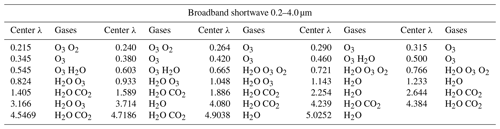Table 8Center wavelengths used for broadband longwave calculations and relevant gases.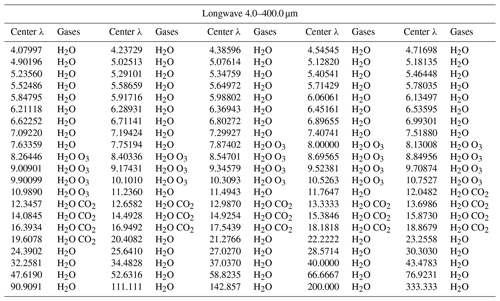The BBR ideal radiances at 250 m resolution as calculated by the radiative transfer code were used to create simulated L1-b data, i.e., BBR filtered radiances (B-NOM) and BBR single-pixel filtered radiances (B-SNG); see the production model in ). This process first involved the convolution of the simulated radiances with the spectral responses of the BBR instrument to obtain broadband SW, LSW, and LW, LLW, radiances at 250 m. Secondly, as the BBR instrument will measure total-wave (TW) radiances, which are not simulated, the TW (LTW) radiances at 250 m resolutions are calculated as the weighted sum of the longwave and shortwave channels (i.e., ${L}_{\mathrm{TW}}={L}_{\mathrm{LW}}+A\cdot {L}_{\mathrm{SW}}$) .

In order to simulate realistic L1 B-SNG inputs, the chopper drum mechanism (CDM) speed has to be taken into account. It has been configured at a 0.7 ratio of the original nominal speed, as recommended to maximize the lifetime of the mechanism, which defines a ground sampling distance for two consecutive SW or TW measurements of 1.1 km. The current BBR simulator software to produce the B-SNG input performs a bilinear interpolation of the 250 m broadband radiances at the positions of the 30-detector array, and this is done for each of the BBR views (fore, nadir and aft). The resulting B-SNG SW and TW radiances for the Halifax scene (Sect. 3.1) are show in Figs. 8 and 9. Finally, a domain integration or point spread function weighting is done to pass from the single-pixel radiances in B-SNG to the nominal BBR resolutions in B-NOM (standard, full and small) sampled every 1 km.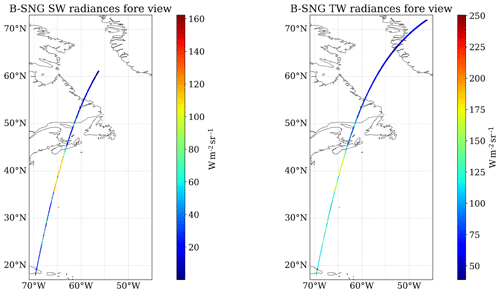Figure 8Simulated B-SNG TOA broadband radiances for the fore view of the BBR SW and TW channels for the Halifax scene. Similar plots are obtained for the nadir and aft views.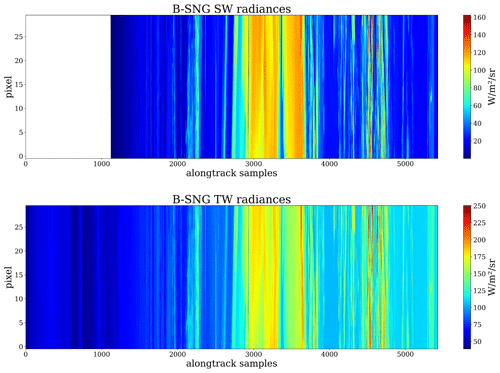Figure 9Simulated B-SNG TOA broadband radiances for the fore view of the BBR SW and TW channels for the Halifax scene.​​​​​​​

3 The test frames

In this section, simulated L1 data for the EarthCARE test scenes are presented and discussed. The level 1 simulated data and various model truth fields for three GEM-derived scenes are available from https://doi.org/10.5281/zenodo.7117115 .

## 3.1 Halifax

The high-latitude part of the Halifax scene features mixed-phase clouds at nighttime, transitioning from deeper clouds with tops up to 6 km around 65 N featuring supercooled liquid in convective cells, to mixed-phase clouds with tops around 3 km at temperatures as cold as −30C, and finally to more broken shallow mixed-phase clouds toward 50 N. Near the center of the frame, a storm system with supercooled layers, convective precipitation, and ice clouds with tops up to altitudes of 13 km is present. South of about 45 N, cloud-free and shallow low-altitude water clouds are present. Also south of 45 N, extended aerosol regions are present. From the ground to above 2 km a marine layer (mainly sea salt) is present and above this a thinner continental pollution layer (mainly fin-mode non-absorbing aerosol) is present. The differences in the lidar ratio associated with these two aerosol regions shown in the middle panel of Fig. 10 is evident.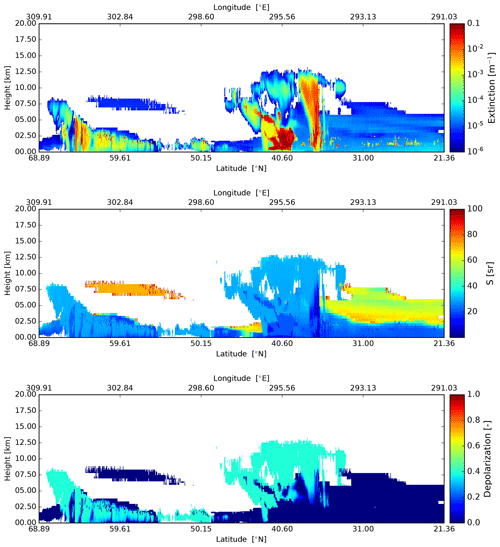Figure 10Cross-sections of the extinction at 355 nm, lidar extinction-to-backscatter ratio, and linear depolarization ratio, following the simulated EarthCARE orbit for the Halifax scene.

The simulated ATLID Mie, Rayleigh, and cross-polar attenuated backscatters are shown in Fig. 11. It can be seen that the lidar penetration into the clouds is limited, as expected, especially in the central part of the frame. However, most of the ice clouds are captured well. The aerosol fields in the southern segment of the frame are also well captured. Quantitative measures of the ability to detect features in the ATLID data are presented in , which describes the ATLID feature mask product (A-FM). The accuracy and sensitivity of quantitative cloud and aerosol extinction and backscatter retrievals applied to the ATLID data are discussed in, e.g.,  and .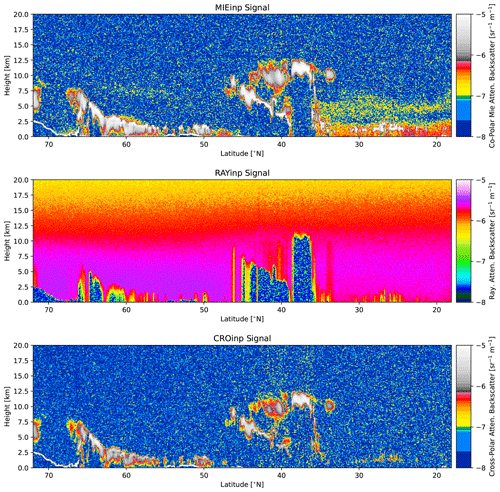Figure 11Simulated Mie channel, Rayleigh channel, and cross-polar ATLID attenuated backscatters for the Halifax scene.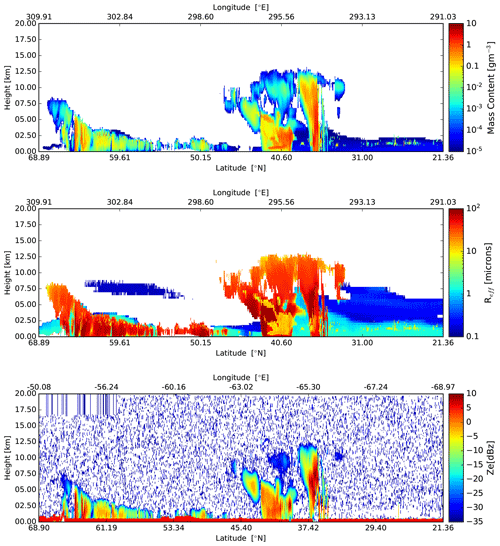Figure 12Cross-sections of the particle mass density, effective particle radius, and simulated observe radar reflectivity, following the simulated EarthCARE orbit for the Halifax scene.

The nadir fields of particle mass, effective radius, and simulated observed radar reflectivity for the full Halifax scene are shown in Fig. 12. The striped area in the radar reflectivity present in the upper-left of the lower panel is due to a simulated change in maximum height covered by the radar, which is associated with a latitude-dependent change in the operating PRF. Radar reflectivity is a strong function of the particle effective radius; hence, in general the larger effective radii regions of the scene are well sampled, while areas containing relativity small water cloud droplets are not.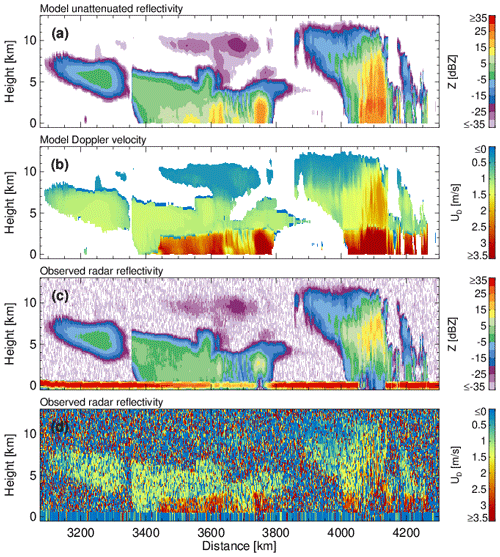Figure 13(a) GEM unattenuated 94 GHz radar reflectivity factor at the model resolution, (b) GEM sedimentation Doppler velocity at the model resolution, (c) CPR raw radar reflectivity factor at the CPR resolution with surface echo, and (d) CPR raw Doppler velocity measurements with no correction applied at the CPR resolution with surface echo.

The GEM model and CPR-simulated signals for a selected region of the Halifax scene are shown in Fig. 13. Due to the large horizontal extent of the scene, we focus on the 30–48 N simulated region that covers the frontal and convective systems. The top two panels shows the unattenuated 94 GHz radar reflectivity and the reflectivity-weighted hydrometeor sedimentation velocity at the GEM grid resolution. The two systems are characterized by high cloud tops (12 km), periods with thick high-level clouds, and extensive periods with light and moderate precipitation. The lower two panels show the raw (uncorrected) CPR radar reflectivity and mean Doppler velocity measurements. The EarthCARE CPR has sufficient sensitivity to detect the hydrometeor layers except weak reflectivity echoes near the highest cloud tops. The strong 94 GHz attenuation by hydrometeors results in missed detections near the surface. This can be clearly seen by the depression of the surface echo radar reflectivity at 3700 km and the complete loss of the surface echo around 4100 km. The faint CPR echoes that fill the surface echo gap around 4100 km are due to multiple scattering . As expected, the CPR raw Doppler velocity field (500 m along-track integration) is noisy . The post-processing algorithm described in is expected to significantly improve the quality of the CPR Doppler velocity measurements. Despite their noisiness, the CPR Doppler velocities reproduce the main features of the GEM model Doppler velocities, namely the transition from solid to liquid hydrometeors and the low-sedimentation Doppler velocities in the upper cloud levels. More details concerning the performance of the CPR and the CPR cloud algorithms are provided in and .

The simulated shortwave TOA radiances for the MSI shortwave channels are shown in Fig. 14. Here, north of about 55 N the Sun is below the horizon so that the radiances are zero. Below about 42 N, the swath is above the ocean with the exception of passing over the Dominican Republic near the southern frame border. The NDVI (normalized difference vegetation index, defined as the difference in reflectances divided by their sum for the two indicated channels) fields corresponding to the radiances shown in Fig. 14 are shown in Fig. 15.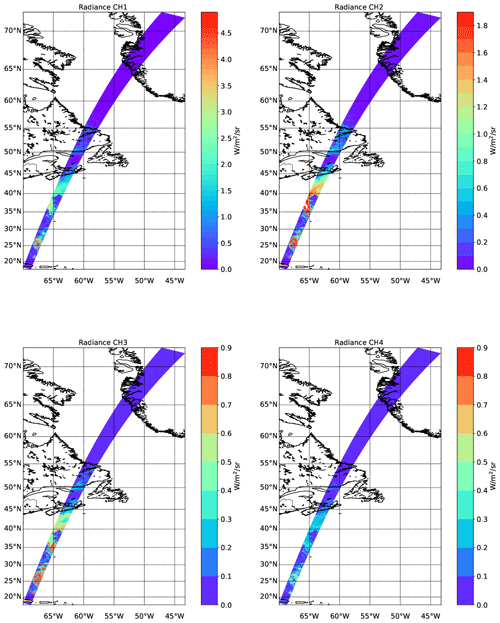Figure 14Simulated TOA radiances for the four SW EarthCARE MSI channels for the Halifax scene.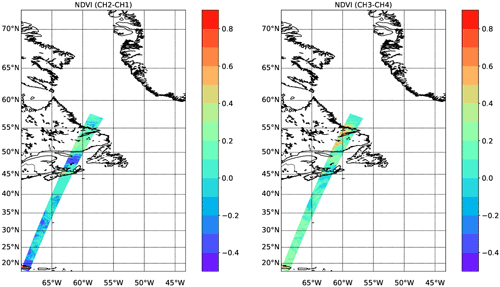Figure 15NDVI values corresponding to the simulated radiances shown in Fig. 14.

The simulated longwave TOA brightness temperatures for the MSI longwave channels are shown in Fig. 16. Here cold (but low-altitude) clouds tops north of 55 N are visible, as are the cold (but high) cloud top temperatures between 35 and 45 N. The warm land and ocean temperatures are also apparent in the mainly cloud-free region south of about 35 N. A close-up of the channel 1 radiances for the Halifax scene between 32.5 and 37.5 N can be seen within Fig. 17.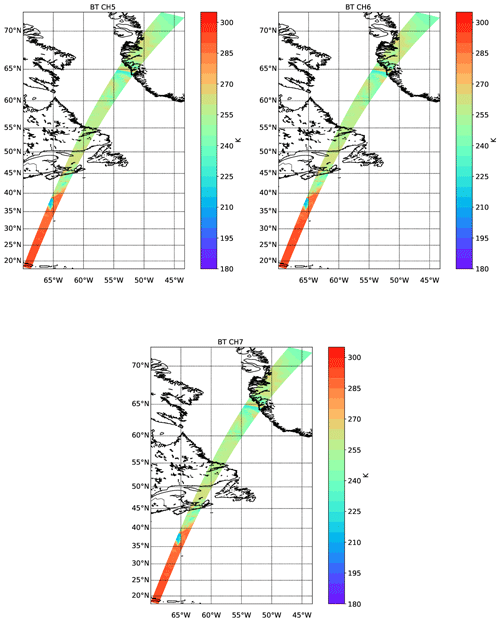Figure 16Simulated TOA brightness temperatures for the three LW EarthCARE MSI channels for the Halifax scene.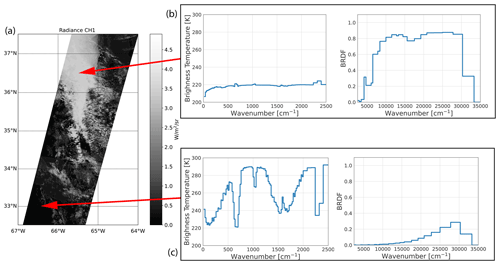Figure 17(a) TOA channel 1 radiances for the Halifax scene between 32.5 and 37.5 N. (b) LW brightness temperatures and SW BRDFs as a function of wavenumber corresponding to a high-altitude thick cloud. (c) LW brightness temperatures and SW BRDFs for each BBR channel corresponding to cloud-free conditions over ocean.

## 3.2 Baja

The Baja scene is the second GEM-derived scene. This scene has a lot of topographical variation compared to the Halifax scene and contains large regions of thinly distributed aerosols. In addition, high-level ice clouds are present south of 35 N. Near the center of the scene, above the Rocky Mountains, extended regions of optically thick ice and water clouds are present.

The extinction, lidar radio, and depolarization model truth fields for the nadir track are shown in Fig. 18. The lidar-observed attenuated backscatter fields are shown in Fig. 19, while the particle mass density, effective radius, and equivalent radar reflectivity are shown in Fig. 20.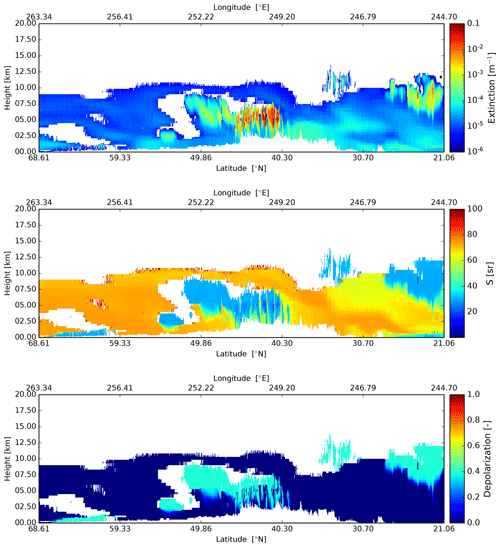Figure 18Cross-sections of the extinction at 355 nm, lidar extinction-to-backscatter ratio, and linear depolarization ratio, following the simulated EarthCARE orbit for the Baja scene.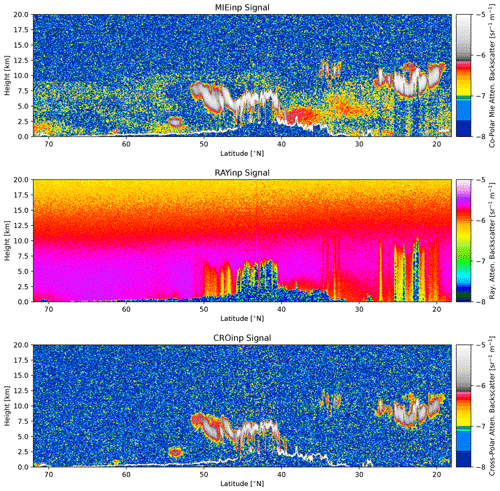Figure 19Simulated Mie channel, Rayleigh channel, and cross-polar ATLID attenuated backscatters for the Baja scene.Figure 20Cross-sections of the particle mass density, effective particle radius, and observed radar reflectivity, following the simulated EarthCARE orbit for the Baja scene.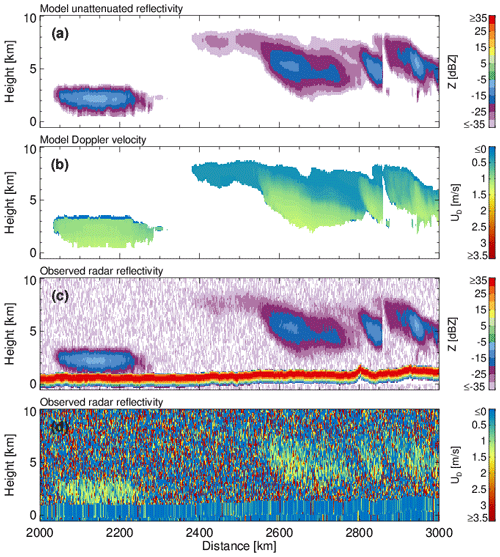Figure 21(a) GEM unattenuated 94 GHz radar reflectivity factor at the model resolution, (b) GEM sedimentation Doppler velocity at the model resolution, (c) CPR raw radar reflectivity factor at the CPR resolution with surface echo, and (d) CPR raw Doppler velocity measurements with no correction applied at the CPR resolution with surface echo.

The GEM model and CPR-simulated signals for a selected region of the Baja scene are shown in Fig. 21. Due to the large horizontal extent of the scene, we focus on the 45–55 N simulated region that covers the northern part of the GEM simulation over the Rockies that includes ice clouds. The top two panels shows the unattenuated 94 GHz radar reflectivity and the reflectivity-weighted hydrometeor sedimentation velocity at the GEM grid resolution. The ice clouds are characterized by low radar reflectivity and Doppler velocities that increase towards the hydrometeor layer base. Due to their low radar reflectivity, a significant fraction of the EarthCARE CPR echoes are close to its detection limit (−35 dBZ). The low radar reflectivities contribute to the noisiness of the CPR raw Doppler velocities.

The simulated shortwave TOA radiances for the MSI shortwave channels are shown in Fig. 22. Here, north of about 55 N snow and ice surfaces are present, leading to high channel 1 and 2 radiances even when clear-sky conditions are present. The snow and ice surfaces also stand out in the CH3-CH4 NDVI images (Fig. 23).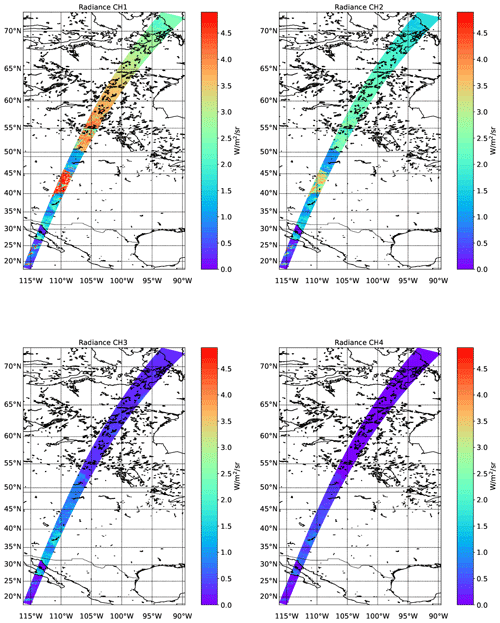Figure 22Simulated TOA radiances for the four SW EarthCARE MSI channels for the Baja scene.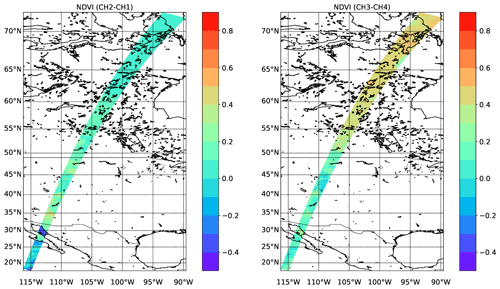Figure 23NDVI values corresponding to the simulated radiances shown in Fig. 22.

The simulated longwave TOA brightness temperatures for the MSI longwave channels are shown in Fig. 24. Here there are cold (but low-altitude) cloud tops and surfaces north of 55 N visible, as well as cold (but high) cloud top temperatures around 45 N. The warm land and ocean temperatures are also apparent in the mainly cloud-free region south of about 40 N.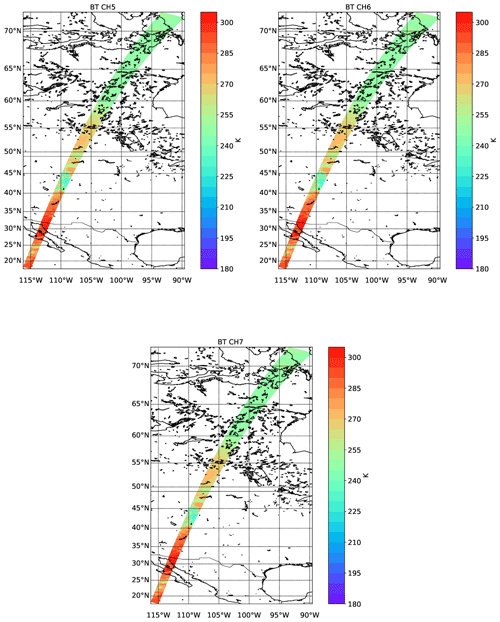Figure 24Simulated TOA brightness temperatures for the three LW EarthCARE MSI channels for the Baja scene.

## 3.3 Hawaii

The third GEM-based scene is the Hawaii scene, situated almost completely over ocean, where the nadir track is completely over ocean and a few of the smaller Hawaiian islands are within the MSI track. This scene exhibits areas of clear sky, upper-level cirrus, and a tropical convective system near the center of the scene. The extinction, lidar ratio, and depolarization model truth fields for the nadir track are shown in Fig. 25. The lidar-observed attenuated backscatter fields are shown in Fig. 26, while the particle mass density, effective radius, and equivalent radar reflectivity are shown in Fig. 27.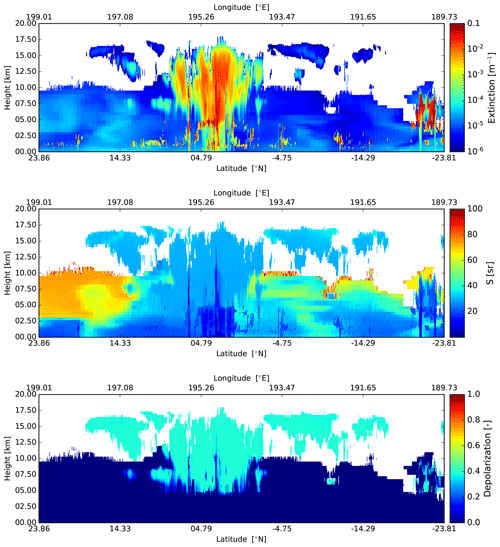Figure 25Cross-sections of the extinction at 355 nm, lidar extinction-to-backscatter ratio, and linear depolarization ratio, following the simulated EarthCARE orbit for the Hawaii scene.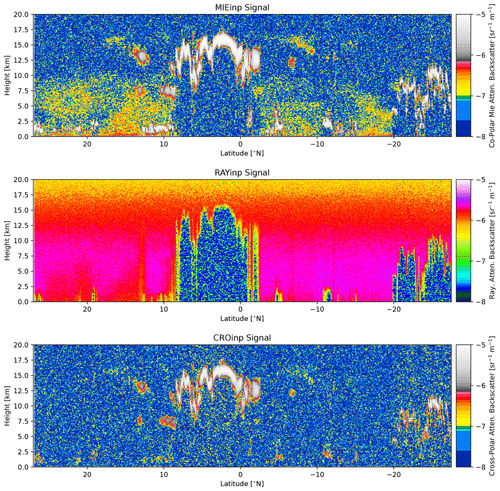Figure 26Simulated Mie channel, Rayleigh channel, and cross-polar ATLID attenuated backscatters for the Hawaii scene.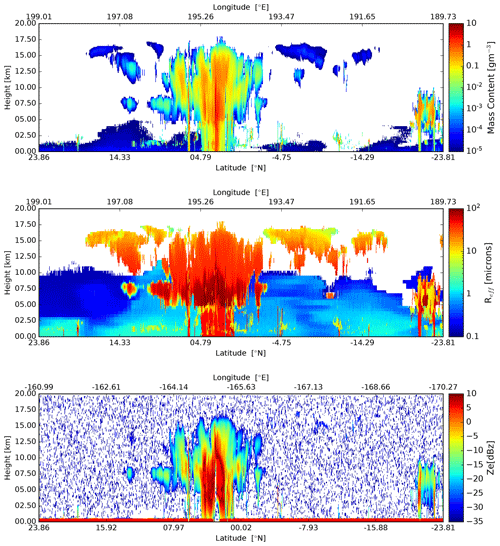Figure 27Cross-sections of the particle mass density, and effective particle radius, and observed Radar reflectivity, following the simulated EarthCARE orbit for the Hawaii scene.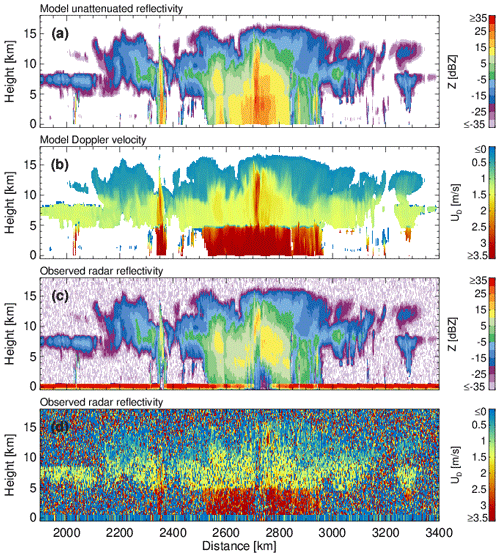Figure 28(a) GEM unattenuated 94 GHz radar reflectivity factor at the model resolution, (b) GEM sedimentation Doppler velocity at the model resolution, (c) CPR raw radar reflectivity factor at the CPR resolution with surface echo, and (d) CPR raw Doppler velocity measurements with no correction applied at the CPR resolution with surface echo.

The GEM model and CPR-simulated signals for the Hawaii scene are shown in Fig. 28. Due to the large horizontal extent of the scene, we focus on the center of the scene where a large tropical convective and stratiform precipitating system was simulated. The top two panels show the unattenuated 94 GHz radar reflectivity and the reflectivity-weighted hydrometeor sedimentation velocity at the GEM grid resolution. The widespread stratiform precipitation is extensive, covering over 500 km, and the precipitation system reaches tops of 16 km. Embedded convective cells are simulated at 2375 and 2750 km along-track distance. The lower two panels show the raw (uncorrected) CPR radar reflectivity and mean Doppler velocity measurements. The EarthCARE CPR has sufficient sensitivity to detect the hydrometeor layers, except for weak reflectivity echoes near the highest cloud tops. The strong 94 GHz attenuation by hydrometeors results in significant attenuation in the rain layer and complete loss of the surface echo return in the two embedded convective cells. Significant multiple scattering is simulated at 2750 km, as seen by the faint CPR echoes that fill the surface echo gap around 4100 km . As expected, the CPR raw Doppler velocity field (500 m along track integration) is noisy . Despite their noisiness, the CPR Doppler velocities reproduce the main features of the GEM model Doppler velocities, namely, the transition from solid to liquid hydrometeors and the low-sedimentation Doppler velocities in the upper cloud levels.

The simulated shortwave TOA radiances for the MSI shortwave channels are shown in Fig. 29. Here the cloud features are all clearly visible against the low-albedo ocean. The NDVI fields are shown in Fig. 30. The high values in the right panel correspond to one of the few land areas (the island of Niihau) within the MSI swath.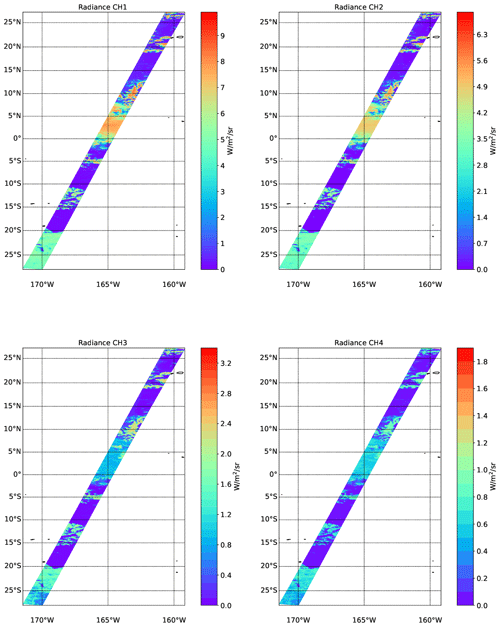Figure 29Simulated TOA radiances for the four SW EarthCARE MSI channels for the Hawaii scene.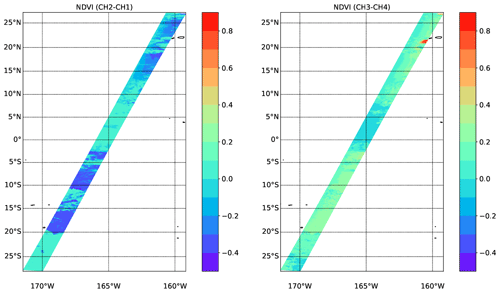Figure 30NDVI values corresponding to the simulated radiances shown in Fig. 29.

The simulated longwave TOA brightness temperatures for the MSI longwave channels are shown in Fig. 31. Here the very cold cloud tops near the center of the scene are especially prominent.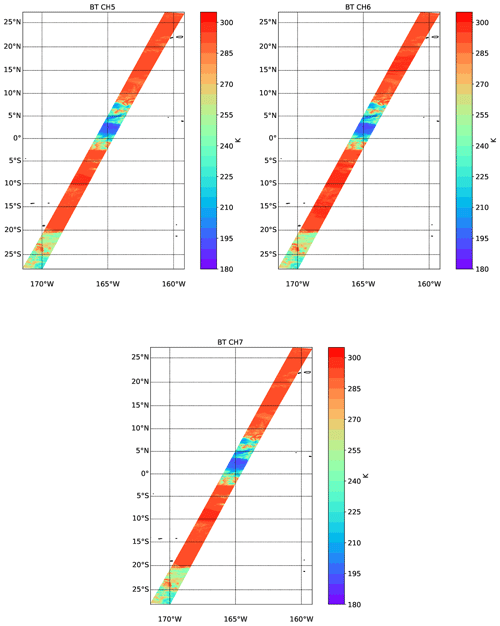Figure 31Simulated TOA brightness temperatures for the three LW EarthCARE MSI channels for the Hawaii scene.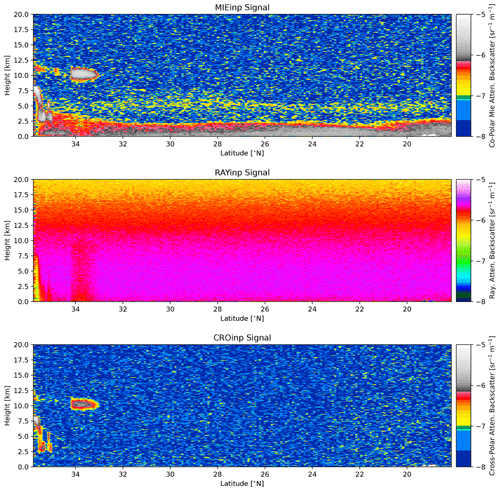Figure 32Simulated Mie channel, Rayleigh channel, and cross-polar ATLID attenuated backscatters for the Halifax aerosol scene.

## 3.4 Halifax aerosol scene

As a last test scene based on GEM and CAMS inputs and used in the processor development within this special issue, a mainly aerosol scene was built from a subsection of the Halifax scene. For this “Halifax aerosol” scene the region south of 36 N was used; however, all liquid clouds and aerosol types were removed except for the coarse-mode non-absorbing aerosol (sea salt). The remaining aerosol particle density was scaled by a factor of 2 to increase the total optical thickness and detectability by the MSI instrument. The resulting scene contains ice cloud north of about 33 N and an aerosol-rich boundary layer marine aerosol layer limited to an altitude of about 2.5 km with a very tenuous layer above. The lidar-observed attenuated backscatter fields are shown in Fig. 32, while the MSI images are shown in Figs. 3335.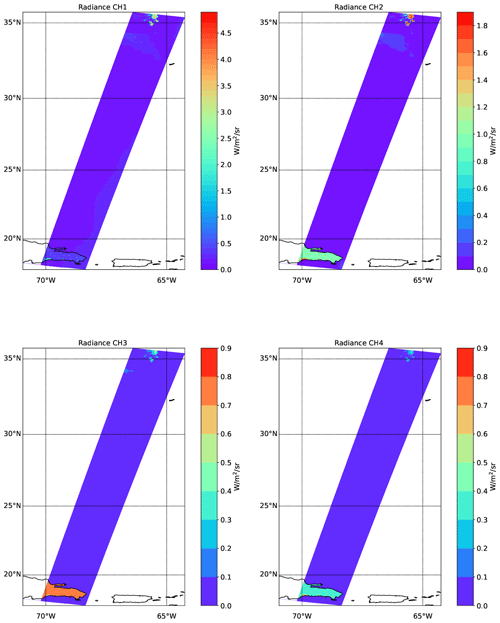Figure 33Simulated TOA radiances for the four SW EarthCARE MSI channels for the Halifax aerosol scene.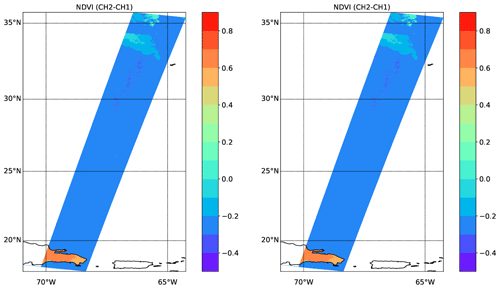Figure 34NDVI values corresponding to the simulated radiances shown in Fig. 33.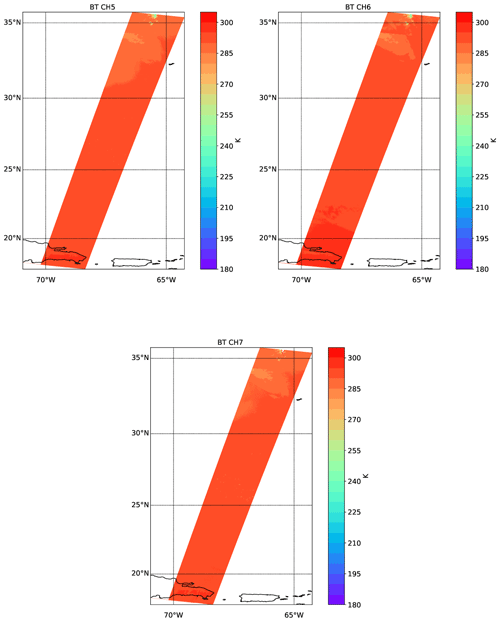Figure 35Simulated TOA brightness temperatures for the three LW EarthCARE MSI channels for the Hawaii scene.

4 Conclusion

In this paper the main testing and development scenes used for EarthCARE L2 algorithm scientific and technical development and testing have been presented. The model data used as the basis for the scenes are discussed in a companion paper . The focus of this paper has been to present the modeled L1 signals for each of the EarthCARE instruments and to document the methods used in their creation. The L1 signals, together with the corresponding model truth field, have in turn been used to develop a suite of single- and multi-instrument retrieval processors. These processors are described by various companion papers. Further insight into the expected precision, accuracy, and coverage of the EarthCARE data can be found in an algorithm inter-comparison paper .

Numerous other studies, beyond what has been presented within this special issue, could be conducted using this simulated data set. Post-launch, the simulations presented here will continue to play a role in the evolution of the various processors. Post-launch activities, including investigating the effects of in-orbit EarthCARE instrument degradation, may be conducted using the present simulations as a base. In addition, the simulations could be expanded to include other past instruments, e.g., CALIPSO, or future proposed lidars or radars. Such activities may be useful in understanding the relationship between, for example, the existing CALIPSO aerosol record and expected EarthCARE results.

The detail, realism, physical consistency, and scale of the test scenes developed for EarthCARE algorithm development, implementation, and testing comprise a unique effort. The creation of detailed realistic test scenes has involved considerable work but should be judged as time well spent. Not only have they served to develop new scientific inversion approaches, but they have also proved very useful in terms of technical development. It is true that the success of any inversion procedure must be evaluated using real data; however, the ability to compare against a “model truth” is invaluable when constructing new inversion algorithms, both in a scientific sense and in a technical (e.g., debugging) sense.

When actual EarthCARE data are available, there will no doubt be surprises to be dealt with. The extensive algorithm development process, aided by the simulations, will help ensure that these unexpected issues will be handled efficiently.

Data availability

The EarthCARE level 2 nadir model truth data and the simulated L1 products discussed in this paper are available from https://doi.org/10.5281/zenodo.7117115 .

Author contributions

All authors of this paper, namely DPD, PK, AVB, and GJvZ, contributed fairly with regard to the development of the studies that led to the results presented here. PK was mainly responsible for writing Sect. 2.4, and AVB was mainly responsible for writing Sect. 2.6. DPD was mainly responsible for writing the remaining sections.

Competing interests

At least one of the (co-)authors is a member of the editorial board of Atmospheric Measurement Techniques. The peer-review process was guided by an independent editor, and the authors also have no other competing interests to declare.

Disclaimer

Publisher's note: Copernicus Publications remains neutral with regard to jurisdictional claims made in the text, published maps, institutional affiliations, or any other geographical representation in this paper. While Copernicus Publications makes every effort to include appropriate place names, the final responsibility lies with the authors.

Special issue statement

Acknowledgements

This work has been funded by ESA grant nos. 22638/09/NL/CT (ATLAS), ESA ITT 1-7879/14/NL/CT (APRIL), and 4000134661/21/NL/AD (CARDINAL). We thank Tobias Wehr, Michael Eisinger, and Anthony Illingworth for valuable discussions and their support for this work over many years. A special acknowledgement is in order for Tobias Wehr, ESA's EarthCARE Mission scientist who unexpectedly passed away recently. Tobias was eagerly looking forward to EarthCARE's launch, a mission to which he had dedicated a considerable span of his career. His support for the science community, collaborative approach, and enthusiasm for the mission science will not be forgotten.

Financial support

This research has been supported by the European Space Agency (ESA; grant nos. 22638/09/NL/CT (ATLAS), ESA ITT 1-7879/14/NL/CT (APRIL), and 4000134661/21/NL/AD (CARDINAL)).

Review statement

This paper was edited by Ulla Wandinger and reviewed by two anonymous referees.

References

Barker, H. and Li, J.: Accelerating Radiative Transfer Calculations for High‐Resolution Atmospheric Models, Q. J. Roy. Meteor. Soc., 145, 2046–2069, https://doi.org/10.1002/qj.3543, 2019. a

Barker, H. W. and Liu, D.: Inferring Optical Depth of Broken Clouds from Landsat Data, J. Climate, 8, 2620–2630, https://doi.org/10.1175/1520-0442(1995)008<2620:IODOBC>2.0.CO;2, 1995. a

Barker, H. W., Cole, J. N. S., Qu, Z., Villefranque, N., and Shephard, M.: Radiative closure assessment of retrieved cloud and aerosol properties for the EarthCARE mission: the ACMB-DF product, Atmos. Meas. Tech., to be submitted, 2023. a

Battaglia, A. and Tanelli, S.: DOMUS: DOppler MUltiple-Scattering Simulator, IEEE T. Geosci. Remote, 49, 442–450, https://doi.org/10.1109/TGRS.2010.2052818, 2011. a

Battaglia, A., Tanelli, S., Kobayashi, S., Zrnic, D., Hogan, R. J., and Simmer, C.: Multiple-scattering in radar systems: A review, J. Quant. Spectrosc. Ra., 111, 917–947, https://doi.org/10.1016/j.jqsrt.2009.11.024, 2010. a, b, c

Battrick, B.: ESA SP-1279(1) – EarthCARE – Earth Clouds, Aerosols and Radiation Explorer, European Space Agency, Tech. rep., 60 pp., ISBN 92-9092-962-6, 2004. a

Baum, B. A., Yang, P., Heymsfield, A. J., Bansemer, A., Cole, B. H., Merrelli, A., Schmitt, C., and Wang, C.: Ice cloud single-scattering property models with the full phase matrix at wavelengths from 0.2 to 100 µm, J. Quant. Spectrosc. Ra., 146, 123–139, https://doi.org/10.1016/j.jqsrt.2014.02.029, 2014. a, b

Brandes, E. A., Zhang, G., and Vivekanandan, J.: Experiments in Rainfall Estimation with a Polarimetric Radar in a Subtropical Environment, J. Appl. Meteorol. Clim., 41, 674–685, https://doi.org/10.1175/1520-0450(2002)041<0674:EIREWA>2.0.CO;2, 2002. a

Bravo-Aranda, J. A., Belegante, L., Freudenthaler, V., Alados-Arboledas, L., Nicolae, D., Granados-Muñoz, M. J., Guerrero-Rascado, J. L., Amodeo, A., D'Amico, G., Engelmann, R., Pappalardo, G., Kokkalis, P., Mamouri, R., Papayannis, A., Navas-Guzmán, F., Olmo, F. J., Wandinger, U., Amato, F., and Haeffelin, M.: Assessment of lidar depolarization uncertainty by means of a polarimetric lidar simulator, Atmos. Meas. Tech., 9, 4935–4953, https://doi.org/10.5194/amt-9-4935-2016, 2016. a

Chang, M.: Overview of the EarthCARE multi-spectral imager and results from the development of the MSI engineering model, in: International Conference on Space Optics – ICSO 2012, Ajaccio, Corse, France, 9–12 October 2012, edited by: Cugny, B., Armandillo, E., and Karafolas, N., International Society for Optics and Photonics, SPIE, 10564, p. 105643Z, https://doi.org/10.1117/12.2551545, 2019. a

Cole, J. N. S., Barker, H. W., Qu, Z., Villefranque, N., and Shephard, M. W.: Broadband radiative quantities for the EarthCARE mission: the ACM-COM and ACM-RT products, Atmos. Meas. Tech., 16, 4271–4288, https://doi.org/10.5194/amt-16-4271-2023, 2023. a

do Carmo, J. P., Hélière, A., Le Hors, L., Toulemont, Y., Lefebvre, A., and Lefebvre, A.: ATLID, ESA Atmospheric LIDAR Developement Status, EPJ Web Conf., 119, 04003, https://doi.org/10.1051/epjconf/201611904003, 2016. a, b

do Carmo, J. P., de Villele, G., Wallace, K., Lefebvre, A., Ghose, K., Kanitz, T., Chassat, F., Corselle, B., Belhadj, T., and Bravetti, P.: ATmospheric LIDar (ATLID): Pre-Launch Testing and Calibration of the European Space Agency Instrument That Will Measure Aerosols and Thin Clouds in the Atmosphere, Atmosphere, 12, 76, https://doi.org/10.3390/atmos12010076, 2021. a, b

Donovan, D., van Zadelhoff, G.-J., and Wang, P.: The EarthCARE lidar cloud and aerosol profile processor: the A-AER, A-EBD, A-TC and A-ICE products, Atmos. Meas. Tech., in preparation, 2023. a

Donovan, D. P., Klein Baltink, H., Henzing, J. S., de Roode, S. R., and Siebesma, A. P.: A depolarisation lidar-based method for the determination of liquid-cloud microphysical properties, Atmos. Meas. Tech., 8, 237–266, https://doi.org/10.5194/amt-8-237-2015, 2015. a, b, c

Eisinger, M., Marnas, F., Wallace, K., Kubota, T., Tomiyama, N., Ohno, Y., Tanaka, T., Tomita, E., Wehr, T., and Bernaerts, D.: The EarthCARE Mission: Science Data Processing Chain Overview, EGUsphere [preprint], https://doi.org/10.5194/egusphere-2023-1998, 2023. a, b, c

Erfani, E. and Mitchell, D. L.: Developing and bounding ice particle mass- and area-dimension expressions for use in atmospheric models and remote sensing, Atmos. Chem. Phys., 16, 4379–4400, https://doi.org/10.5194/acp-16-4379-2016, 2016. a, b, c

Fell, F. and Fischer, J.: Numerical simulation of the light field in the atmosphere–ocean system using the matrix-operator method, J. Quant. Spectrosc. Ra., 69, 351–388, https://doi.org/10.1016/S0022-4073(00)00089-3, 2001. a

Grenfell, T. C. and Warren, S. G.: Representation of a nonspherical ice particle by a collection of independent spheres for scattering and absorption of radiation, J. Geophys. Res.-Atmos., 104, 31697–31709, https://doi.org/10.1029/1999JD900496, 1999. a

Hale, G. M. and Querry, M. R.: Optical Constants of Water in the 200-nm to 200-µm Wavelength Region, Appl. Optics, 12, 555–563, https://doi.org/10.1364/AO.12.000555, 1973. a

Haynes, J. M., L'Ecuyer, T. S., Stephens, G. L., and Jakob, C.: Cloud and precipitation regimes revealed by combined CloudSat and ISCCP observations, AGU Fall Meeting Abstracts, abstract id. A44B-04, http://adsabs.harvard.edu/abs/2009AGUFM.A44B..04H (last access: 19 October 2023), 2009. a

Hogan, R. J. and Battaglia, A.: Fast Lidar and Radar Multiple-Scattering Models. Part II: Wide-Angle Scattering Using the Time-Dependent Two-Stream Approximation, J. Atmos. Sci., 65, 3636–3651, https://doi.org/10.1175/2008JAS2643.1, 2008. a

Hogan, R. J. and Westbrook, C. D.: Equation for the Microwave Backscatter Cross Section of Aggregate Snowflakes Using the Self-Similar Rayleigh-Gans Approximation, J. Atmos. Sci., 71, 3292–3301, https://doi.org/10.1175/JAS-D-13-0347.1, 2014. a

Hogan, R. J., Honeyager, R., Tyynelä, J., and Kneifel, S.: Calculating the millimetre-wave scattering phase function of snowflakes using the self-similar Rayleigh-Gans Approximation, Q. J. Roy. Meteor. Soc., 143, 834–844, https://doi.org/10.1002/qj.2968, 2017. a

Hollstein, A. and Fischer, J.: Radiative transfer solutions for coupled atmosphere ocean systems using the matrix operator technique, J. Quant. Spectrosc. Ra., 113, 536–548, https://doi.org/10.1016/j.jqsrt.2012.01.010, 2012. a

Hostetler, C. A., Behrenfeld, M. J., Hu, Y., Hair, J. W., and Schulien, J. A.: Spaceborne Lidar in the Study of Marine Systems, Annu. Rev. Mar. Sci., 10, 121–147, https://doi.org/10.1146/annurev-marine-121916-063335, 2018. a

Hünerbein, A., Bley, S., Horn, S., Deneke, H., and Walther, A.: Cloud mask algorithm from the EarthCARE Multi-Spectral Imager: the M-CM products, Atmos. Meas. Tech., 16, 2821–2836, https://doi.org/10.5194/amt-16-2821-2023, 2023. a

Illingworth, A. J., Barker, H. W., Beljaars, A., Ceccaldi, M., Chepfer, H., Clerbaux, N., Cole, J., Delanoë, J., Domenech, C., Donovan, D. P., Fukuda, S., Hirakata, M., Hogan, R. J., Huenerbein, A., Kollias, P., Kubota, T., Nakajima, T., Nakajima, T. Y., Nishizawa, T., Ohno, Y., Okamoto, H., Oki, R., Sato, K., Satoh, M., Shephard, M. W., Velázquez-Blázquez, A., Wandinger, U., Wehr, T., and van Zadelhoff, G.-J.: The EarthCARE Satellite: The Next Step Forward in Global Measurements of Clouds, Aerosols, Precipitation, and Radiation, B. Am. Meteorol. Soc., 96, 1311–1332, https://doi.org/10.1175/BAMS-D-12-00227.1, 2015. a

Inness, A., Ades, M., Agustí-Panareda, A., Barré, J., Benedictow, A., Blechschmidt, A.-M., Dominguez, J. J., Engelen, R., Eskes, H., Flemming, J., Huijnen, V., Jones, L., Kipling, Z., Massart, S., Parrington, M., Peuch, V.-H., Razinger, M., Remy, S., Schulz, M., and Suttie, M.: The CAMS reanalysis of atmospheric composition, Atmos. Chem. Phys., 19, 3515–3556, https://doi.org/10.5194/acp-19-3515-2019, 2019. a

Josset, D., Tanelli, S., Hu, Y., Pelon, J., and Zhai, P.: Analysis of Water Vapor Correction for CloudSat W-Band Radar, IEEE T. Geosci. Remote, 51, 3812–3825, https://doi.org/10.1109/TGRS.2012.2228659, 2013. a

Kato, S., Ackerman, T. P., Mather, J. H., and Clothiaux, E. E.: The k-distribution method and correlated-k approximation for a shortwave radiative transfer model, J. Quant. Spectrosc. Ra., 62, 109–121, https://doi.org/10.1016/S0022-4073(98)00075-2, 1999. a

Kollias, P., Albrecht, B. A., and Marks, F.: Why Mie?, B. Am. Meteorol. Soc., 83, 1471–1483, https://doi.org/10.1175/BAMS-83-10-1471, 2002. a

Kollias, P., Clothiaux, E. E., Miller, M. A., Albrecht, B. A., Stephens, G. L., and Ackerman, T. P.: Millimeter-Wavelength Radars: New Frontier in Atmospheric Cloud and Precipitation Research, B. Am. Meteorol. Soc., 88, 1608–1624, https://doi.org/10.1175/BAMS-88-10-1608, 2007. a

Kollias, P., Tanelli, S., Battaglia, A., and Tatarevic, A.: Evaluation of EarthCARE Cloud Profiling Radar Doppler Velocity Measurements in Particle Sedimentation Regimes, J. Atmos. Ocean. Tech., 31, 366–386, https://doi.org/10.1175/JTECH-D-11-00202.1, 2014. a, b, c, d, e

Kollias, P., Battaglia, A., Lamer, K., Treserras, B. P., and Braun, S. A.: Mind the Gap - Part 3: Doppler Velocity Measurements From Space, Frontiers in Remote Sensing, 3, 860284, https://doi.org/10.3389/frsen.2022.860284, 2022. a, b

Kollias, P., Puidgomènech Treserras, B., Battaglia, A., Borque, P. C., and Tatarevic, A.: Processing reflectivity and Doppler velocity from EarthCARE's cloud-profiling radar: the C-FMR, C-CD and C-APC products, Atmos. Meas. Tech., 16, 1901–1914, https://doi.org/10.5194/amt-16-1901-2023, 2023. a, b, c, d

Lamer, K., Kollias, P., Battaglia, A., and Preval, S.: Mind the gap – Part 1: Accurately locating warm marine boundary layer clouds and precipitation using spaceborne radars, Atmos. Meas. Tech., 13, 2363–2379, https://doi.org/10.5194/amt-13-2363-2020, 2020. a

Li, L., Heymsfield, G. M., Tian, L., and Racette, P. E.: Measurements of Ocean Surface Backscattering Using an Airborne 94-GHz Cloud Radar—Implication for Calibration of Airborne and Spaceborne W-Band Radars, J. Atmos. Ocean. Tech., 22, 1033–1045, https://doi.org/10.1175/JTECH1722.1, 2005. a

Lin, Z., Stamnes, S. A., Jin, Z., Laszlo, I., Tsay, S.-C., Wiscombe, W. J., and Stamnes, K.: Improved discrete ordinate solutions in the presence of an anisotropically reflecting lower boundary: Upgrades of the DISORT computational tool, J. Quant. Spectrosc. Ra., 157, 119–134, 2015. a

Mason, S. L., Cole, J. N. S., Docter, N., Donovan, D. P., Hogan, R. J., Hünerbein, A., Kollias, P., Puigdomènech Treserras, B., Qu, Z., Wandinger, U., and van Zadelhoff, G.-J.: An intercomparison of EarthCARE cloud, aerosol and precipitation retrieval products, EGUsphere [preprint], https://doi.org/10.5194/egusphere-2023-1682, 2023. a, b

Miles, R. B., Lempert, W. R., and Forkey, J. N.: Laser Rayleigh scattering, Meas. Sci. Technol., 12, R33–R51, https://doi.org/10.1088/0957-0233/12/5/201, 2001. a

Mitchell, D. L. and Heymsfield, A. J.: Refinements in the Treatment of Ice Particle Terminal Velocities, Highlighting Aggregates, J. Atmos. Sci., 62, 1637–1644, https://doi.org/10.1175/JAS3413.1, 2005. a

Moody, E. G., King, M. D., Schaaf, C. B., Hall, D. K., and Platnick, S.: Northern Hemisphere five-year average (2000–2004) spectral albedos of surfaces in the presence of snow: Statistics computed from Terra MODIS land products, Remote Sens. Environ., 111, 337–345, https://doi.org/10.1016/j.rse.2007.03.026, 2007. a

Mroz, K., Treserras, B. P., Battaglia, A., Kollias, P., Tatarevic, A., and Tridon, F.: Cloud and precipitation microphysical retrievals from the EarthCARE Cloud Profiling Radar: the C-CLD product, Atmos. Meas. Tech., 16, 2865–2888, https://doi.org/10.5194/amt-16-2865-2023, 2023. a

Pan, X., Shneider, M. N., and Miles, R. B.: Coherent Rayleigh-Brillouin Scattering, Phys. Rev. Lett., 89, 183001, https://doi.org/10.1103/PhysRevLett.89.183001, 2002. a

Qu, Z., Donovan, D. P., Barker, H. W., Cole, J. N. S., Shephard, M. W., and Huijnen, V.: Numerical Model Generation of Test Frames for Pre-launch Studies of EarthCARE’s Retrieval Algorithms and Data Management System, Atmos. Meas. Tech. Discuss. [preprint], https://doi.org/10.5194/amt-2022-300, in review, 2022. a, b, c, d, e, f, g, h

Rosenkranz, P. W.: Water vapor microwave continuum absorption: A comparison of measurements and models, Radio Sci., 33, 919–928, https://doi.org/10.1029/98RS01182, 1998. a, b, c

Saleh, B. E. A. and Teich, M. C.: Fundamentals of photonics; 1st edn., Wiley, New York, NY, https://cds.cern.ch/record/244535 (last access: 17 October 2023), 1991. a

Schaaf, C. B., Gao, F., Strahler, A. H., Lucht, W., Li, X., Tsang, T., Strugnell, N. C., Zhang, X., Jin, Y., Muller, J.-P., Lewis, P., Barnsley, M., Hobson, P., Disney, M., Roberts, G., Dunderdale, M., Doll, C., d'Entremont, R. P., Hu, B., Liang, S., Privette, J. L., and Roy, D.: First operational BRDF, albedo nadir reflectance products from MODIS, Remote Sens. Environ., 83, 135–148, https://doi.org/10.1016/S0034-4257(02)00091-3, 2002. a

Tanelli, S., Durden, S. L., Im, E., Pak, K. S., Reinke, D. G., Partain, P., Haynes, J. M., and Marchand, R. T.: CloudSat's cloud profiling radar after two years in orbit: performance, calibration, and processing, IEEE T. Geosci. Remote, 46, 3560–3573, https://doi.org/10.1109/TGRS.2008.2002030, 2008. a, b

van Zadelhoff, G.-J., Barker, H. W., Baudrez, E., Bley, S., Clerbaux, N., Cole, J. N. S., de Kloe, J., Docter, N., Domenech, C., Donovan, D. P., Dufresne, J.-L., Eisinger, M., Fischer, J., García-Marañón, R., Haarig, M., Hogan, R. J., Hünerbein, A., Kollias, P., Koopman, R., Madenach, N., Mason, S. L., Preusker, R., Puigdomènech Treserras, B., Qu, Z., Ruiz-Saldaña, M., Shephard, M., Velázquez-Blazquez, A., Villefranque, N., Wandinger, U., Wang, P., and Wehr, T.: EarthCARE level-2 demonstration products from simulated scenes, Zenodo [data set], https://doi.org/10.5281/zenodo.7117115, 2023a. a, b

van Zadelhoff, G.-J., Donovan, D. P., and Wang, P.: Detection of aerosol and cloud features for the EarthCARE lidar ATLID: the A-FM product, EGUsphere [preprint], https://doi.org/10.5194/egusphere-2023-145, 2023b. a

Velázquez Blázquez, A., Baudrez, E., Clerbaux, N., and Domenech, C.: Unfiltering of the EarthCARE Broadband Radiometer (BBR) observations: the BM–RAD product, Atmos. Meas. Tech. Discuss. [preprint], https://doi.org/10.5194/amt-2023-170, in review, 2023. a

Vidot, J. and Borbáas, E.: Land surface VIS/NIR BRDF atlas for RTTOV-11: model and validation against SEVIRI land SAF albedo product, Q. J. Roy. Meteor. Soc., 140, 2186–2196, https://doi.org/10.1002/qj.2288, 2014. a

Wandinger, U., Floutsi, A. A., Baars, H., Haarig, M., Ansmann, A., Hünerbein, A., Docter, N., Donovan, D., van Zadelhoff, G.-J., Mason, S., and Cole, J.: HETEAC – The Hybrid End-To-End Aerosol Classification model for EarthCARE, EGUsphere [preprint], https://doi.org/10.5194/egusphere-2022-1241, 2022. a

Witschas, B.: Analytical model for Rayleigh–Brillouin line shapes in air, Appl. Optics, 50, 267–270, https://doi.org/10.1364/AO.50.000267, 2011. a

Zhou, C. and Yang, P.: Backscattering peak of ice cloud particles, Opt. Express, 23, 11995–12003, https://doi.org/10.1364/OE.23.011995, 2015.  a, b, c

Zrnic, D. S.: Simulation of weatherlike Doppler spectra and signal, J. Appl. Meteorol., 14, 619–620, 1975. a

Zrnic, D. S.: Spectral moment estimates from correlated pulse pairs, IEEE T. Aero. Elec. Sys., AES-13, 344–354, 1977. a

It should be noted that The ECSIM framework was used as a basis for the development of a related framework called E3SIM. Within the context of this special issue the term E3SIM may be used in place of E3SIM. In this paper, the framework is referred to as ECSIM, and the focus is not on the framework but rather the models and methods applied to the GEM/CAMS model inputs in order to generate simulated EarthCARE L1 data are described.